# The WDD test with different area sizes

So I have two prior examples of weighting the WDD test (a simple test for pre-post crime counts in an experimental setting):

And a friend recently asked about weighting for different areas, so the test is crime reduction per area density instead of overall counts. First before I get into the example, this isn’t per se necessary. All that matters in the end for this test to be valid is 1) the crime data are Poisson distributed, 2) the control areas follow parallel trends to the treated area. So based on this I’ve advocated that it is ok to have a control area be ‘the rest of the city’ for example.

Some of my work on long term crime trends at micro places, shows low-crime and high-crime areas all tend to follow the same overall temporal trends (and Martin Andresen’s related work one would come to the same conclusion). So that would suggest you can aggregate up many low crimes to make a reasonable control comparison to a hot spot treated area.

So as I will show weighting by area is possible, but it actually changes the identification strategy slightly (whereas the prior two weighting examples do not) – the parallel trends assumption needs to be on the crime per area estimate, as opposed to the original count scale. Since the friend who asked about this is an Excel GURU (check out Grant’s very nice YouTube videos for crime analysis) I will show how to do the calculations in Excel, as well as how to do a simulation to show my estimator behaves as it should. (And the benefit of that is you can do power analysis based on the simulations.)

# Example Calculations in Excel

I have posted an Excel spreadsheet to show the calculations here. But for a quick overview, I made the spreadsheet very similar to the original WDD calculation, you just need to insert your areas for the different treated/control/displacement areas.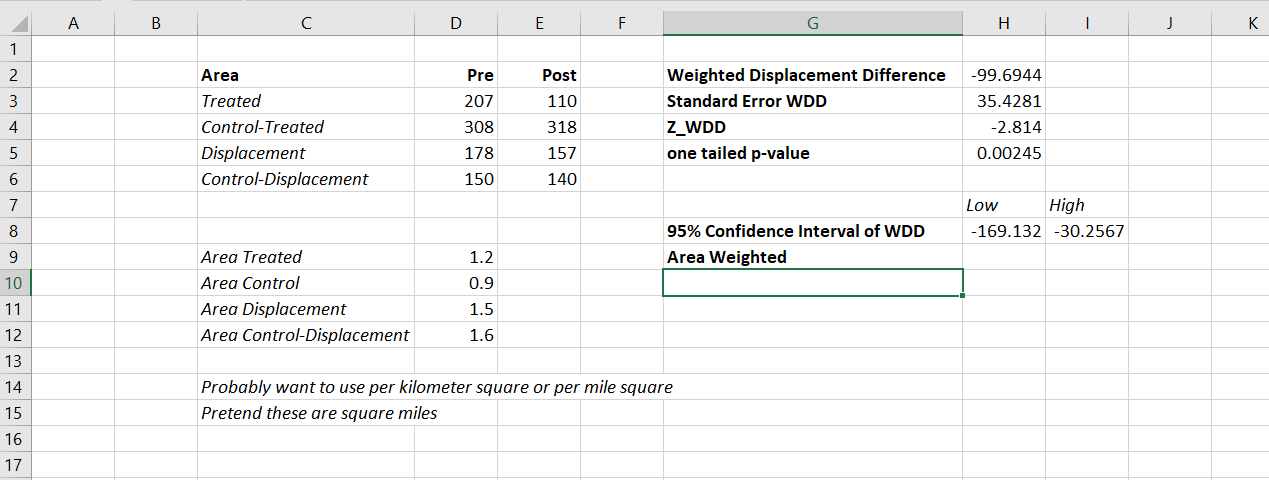And you can check out the formulas, again it is just weighting the estimator by the areas, and then making the appropriate transformations to the variance estimates.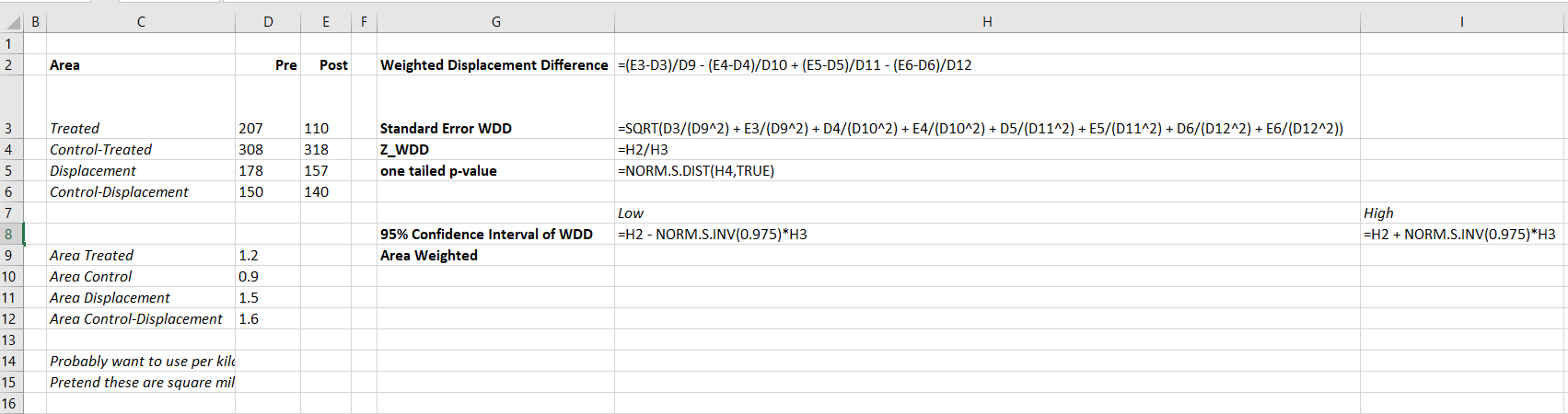I have an added extra portion of this though – a simulation tab to show the estimator works.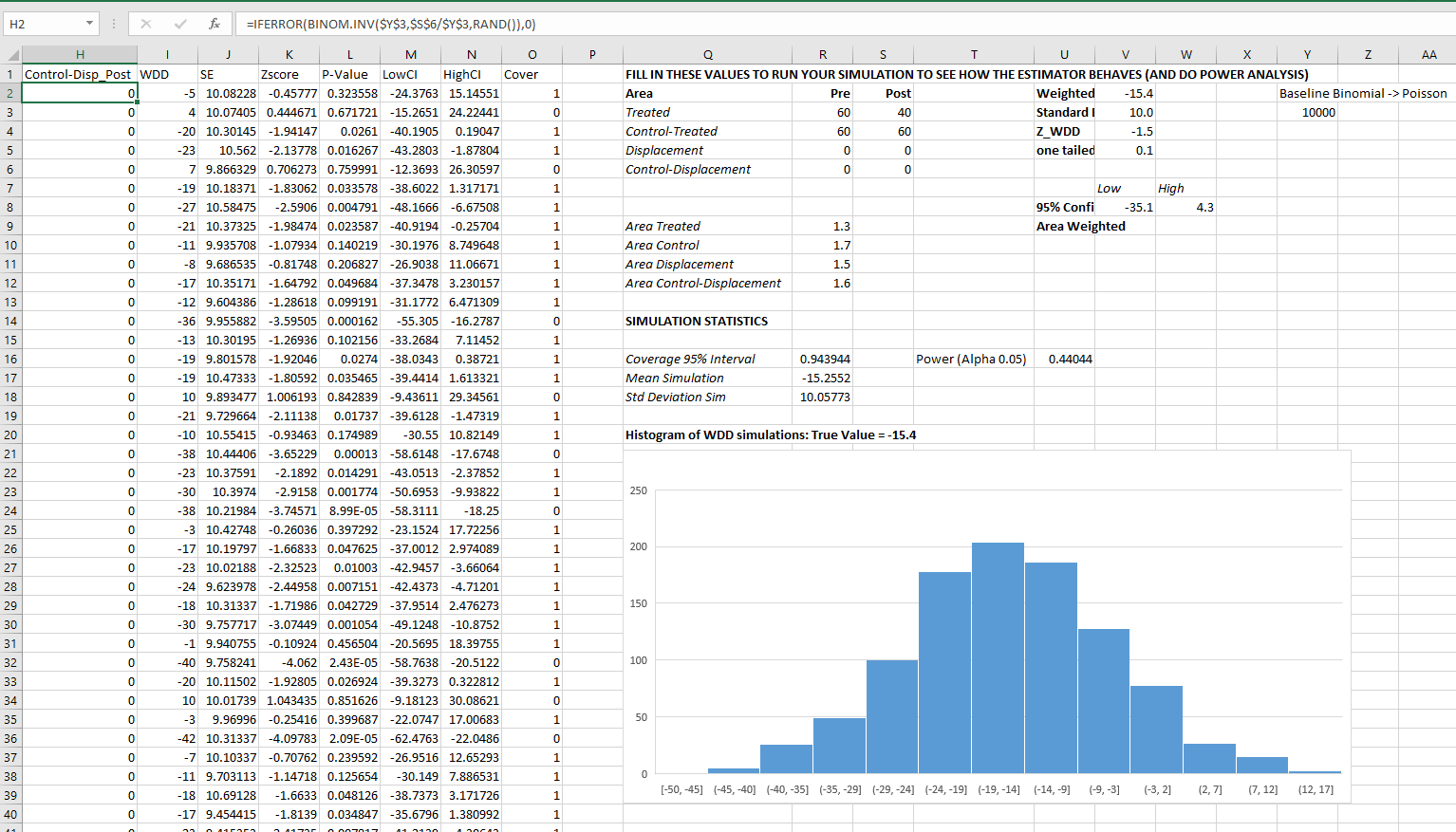Only thing to note, a way to simulate to Poisson data in Excel is to generate a random number on the unit interval `(0,1)`, and then for the distribution of interest use the inverse CDF function. There is no inverse Poisson function in Excel, but you can reasonably approximate it via the inverse binomial with a very large number of trials. I’ve tested and it is good enough for my purposes to use a base of 10k for the binomial trials.

The simulation tab on this spreadsheet you can input your own numbers for planning purposes as well. So the idea is if you think you can only reasonably reduce crimes by X amount in your targeted areas, this lets you do power analysis. So in this example, going from 60 to 40 crimes results in a power estimate of only 0.44 (so you will fail to reject the null over 5 out of 10 times, even if your intervention actually works as well as you think). But if you think you can reduce crimes from 60 to 30, the power in this example gets close to 0.8 (what you typically shoot for in up-front experiments, although there is no harm for going for higher power!). So if you have low power you may want to expand the time periods under study or expand the number of treated areas.

# Wrap Up

Between this and the prior WDD examples, I have about wrapped up all the potential permutations of weighting I can think of offhand. So you can mix/match all of these different weighting strategies together (e.g. you could do multiple time periods and area weighting). It is just algebra and carrying through the correct changes to the variance estimates.

I do have one additional blog post slated in the future. David Wilson has a recent JQC article using a different estimate, but essentially the same pre/post data I am using here. The identifying assumptions are different again for this (parallel trends on the ratio scale, not the linear scale), and I will have more to say when I think you would prefer the WDD to David’s estimator. (In short I think David’s is good for meta-analysis, but I prefer my WDD for individual evaluations.)

# The spatial dispersion of NYC shootings in 2020

If you had asked me at the start of widespread Covid lockdown measures what the effect would be on crime, I am pretty sure I would have guessed it will make crime go down. Fewer people out and about causes fewer interactions that can lead to a crime. That isn’t how it has shaped up though, quite a few places have seen increases in serious violent crime. One of the most dramatic examples of this is that shootings in NYC doubled from 900 in 2019 to over 1800 in 2020. I am going to show how to generate this chart later via some R code, but it is easier to show than to say. NYPD’s open data on shootings (historical, current) go back to 2006.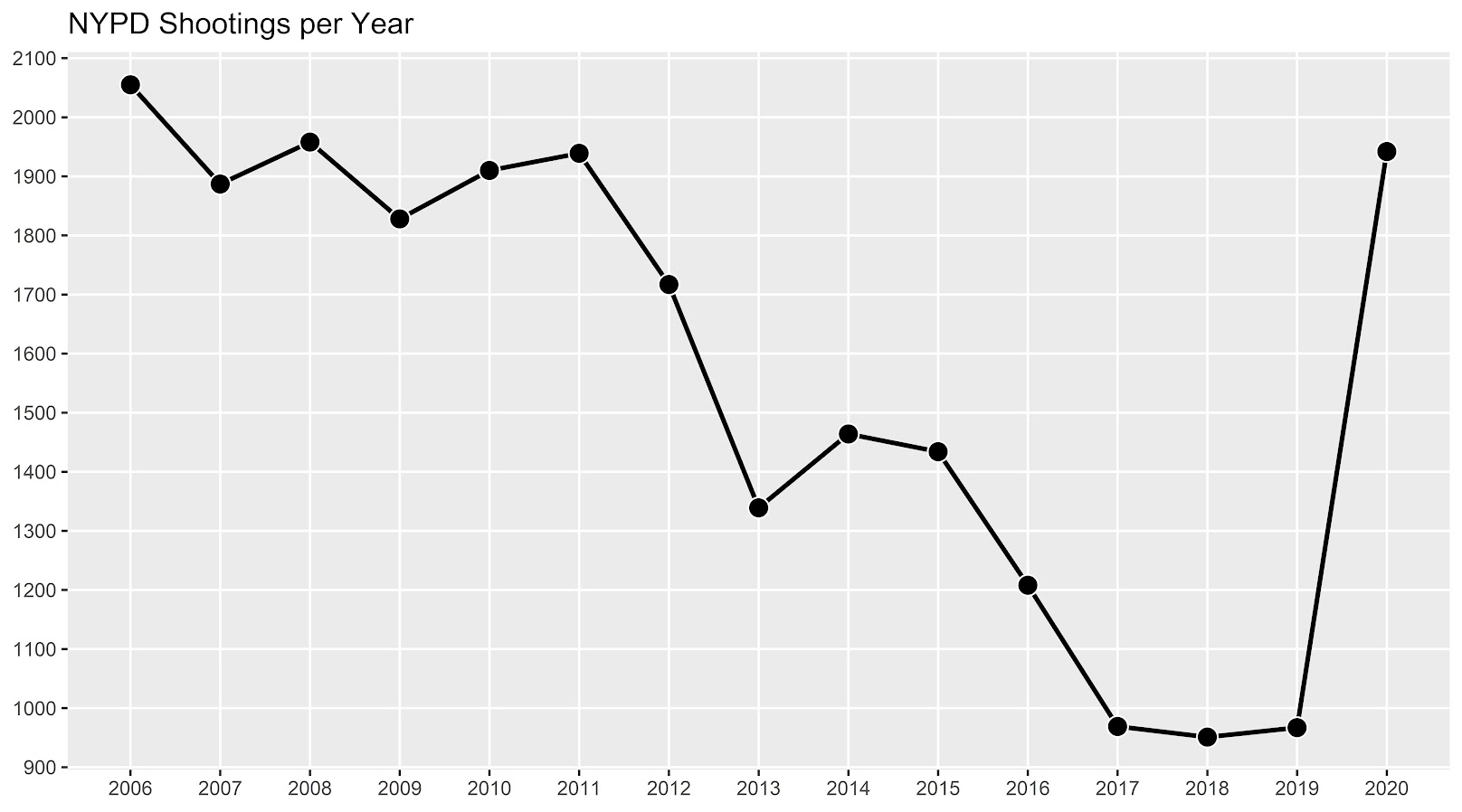I know I am critical on this site of folks overinterpreting crime increases, for example going from 20 to 35 is pretty weak evidence of an increase given the inherent variance for low count Poisson data (a Poisson e-test has a p-value of 0.04 in that case). But going from 900 to 1800 is a much clearer signal.

Jerry Ratcliffe recently posted an R library to do his crime dispersion analysis, so I figured this would be an excellent example use case. The idea behind this analysis is spatial – we know there is a crime increase, but did the increase happen everywhere, or did it just happen in a few locations. Here I am going to use the NYPD shooting data aggregated at the precinct level to test this.

As another note, while I often use micro-spatial units of analysis in my work, this method, along with others (such as the sppt test), are just not going to work out for very low count, very tiny spatial units of analysis. I would suggest offhand to only do this analysis if the spatial units of analysis under study have an average of at least 10 crimes per area in the pre time period. Which is right about on the mark for the precinct analysis in NYC.

Here is the data and R code to follow along, below I will give a walkthrough.

# Crime increase dispersion analysis in R

So first as some front matter, I load in my libraries (Jerry’s `crimedispersion` you can install from github via devtools, see his page for an example), and the function I define here I’ve gone over in a prior blog post of mine as well.

``````###############################
library(ggplot2)
library(crimedispersion)

# Increase contours, see https://andrewpwheeler.com/2020/02/21/some-additional-plots-to-go-with-crime-increase-dispersion/
make_cont <- function(pre_crime,post_crime,levels=c(-3,0,3),lr=10,hr=max(pre_crime)*1.05,steps=1000){
#calculating the overall crime increase
ov_inc <- sum(post_crime)/sum(pre_crime)
#Making the sequence on the square root scale
gr <- seq(sqrt(lr),sqrt(hr),length.out=steps)^2
cont_data <- expand.grid(gr,levels)
names(cont_data) <- c('x','levels')
cont_data\$inc <- cont_data\$x*ov_inc
cont_data\$lines <- cont_data\$inc + cont_data\$levels*sqrt(cont_data\$inc)
return(as.data.frame(cont_data))
}

my_dir <- 'D:\\Dropbox\\Dropbox\\Documents\\BLOG\\NYPD_ShootingIncrease\\Analysis'
setwd(my_dir)
###############################``````

Now we are ready to import our data and stack them into a new data frame. (These are individual incident level shootings, not aggregated. If I ever get around to it I will do an analysis of fatality and distance to emergency rooms like I did with the Philly data.)

``````###############################
# Get the NYPD data and stack it
# From https://data.cityofnewyork.us/Public-Safety/NYPD-Shooting-Incident-Data-Year-To-Date-/5ucz-vwe8
# And https://data.cityofnewyork.us/Public-Safety/NYPD-Shooting-Incident-Data-Historic-/833y-fsy8
# On 2/1/2021
old <- read.csv('NYPD_Shooting_Incident_Data__Historic_.csv', stringsAsFactors=FALSE)
new <- read.csv('NYPD_Shooting_Incident_Data__Year_To_Date_.csv', stringsAsFactors=FALSE)

# Just one column off
print( cbind(names(old), names(new)) )
names(new) <- names(old)
shooting <- rbind(old,new)
###############################``````

Now we just want to do aggregate counts of these shootings per year and per precinct. So first I substring out the year, then use `table` to get aggregate counts in R, then make my nice time series graph using ggplot.

``````###############################
# Create the current year and aggregate
shooting\$Year <- substr(shooting\$OCCUR_DATE, 7, 10)
year_stats <- as.data.frame(table(shooting\$Year))
year_stats\$Year <- as.numeric(as.character(year_stats\$Var1))
year_plot <- ggplot(data=year_stats, aes(x=Year,y=Freq)) +
geom_line(size=1) + geom_point(shape=21, colour='white', fill='black', size=4) +
scale_y_continuous(breaks=seq(900,2100,by=100)) +
scale_x_continuous(breaks=2006:2020) +
theme(axis.title.x=element_blank(), axis.title.y=element_blank(),
panel.grid.minor = element_blank()) +
ggtitle("NYPD Shootings per Year")

year_plot
# Not quite the same as Petes, https://copinthehood.com/shooting-in-nyc-2020/
###############################``````Part of the reason I do this is not because I don’t trust Pete’s analysis, but because I don’t want to embed pictures from someone elses website! So wanted to recreate the time series graph myself. So next up we need to do the same aggregating, but not for the whole city, but by each precinct. You can use the same table method again, but simply pass in additional columns. That gets you the data in long format, so then I reshape it to wide for later analysis (so each row is a single precinct and each column is a yearly count of shootings). (Note there have been some splits in precincts over the years IIRC, I don’t worry about that here, will cause it to be 0,0 in the 2019/2020 data I look at.)

``````###############################
#Now aggregating to year and precinct
counts <- as.data.frame(table(shooting\$Year, shooting\$PRECINCT))
names(counts) <- c('Year','PCT','Count')
# Reshape long to wide
count_wide <-  reshape(counts, idvar = "PCT", timevar = "Year", direction = "wide")
###############################``````

And now we can give Jerry’s package a test run, where you just pass it your variable names.

``````# Jerrys function for crime increase dispersion
output <- crimedispersion(count_wide, 'PCT', 'Count.2019', 'Count.2020')
output``````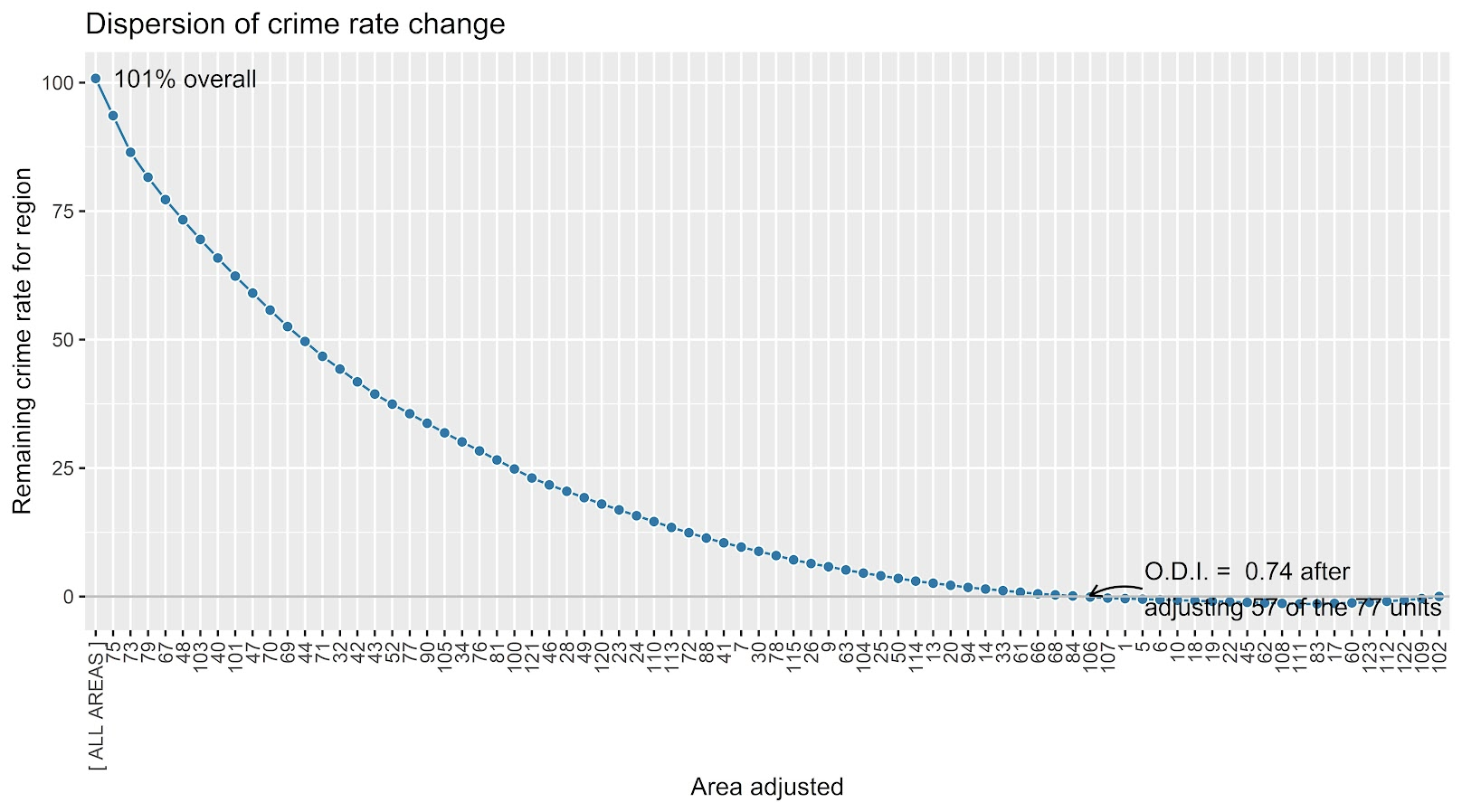The way to understand this is in a hypothetical world in which we could reduce shootings in one precinct at a time, we would need to reduce shootings in 57 of the 77 precincts to reduce 2020 shootings to 2019 levels. So this suggests very widespread increases, it isn’t just concentrated among a few precincts.

Another graph I have suggested to explore this, while taking into account the typical variance with Poisson count data, is to plot the pre crime counts on the X axis, and the post crime counts on the Y axis.

``````###############################
# My example contour with labels
cont_lev <- make_cont(count_wide\$Count.2019, count_wide\$Count.2020, lr=5)

eq_plot <- ggplot() +
geom_line(data=cont_lev, color="darkgrey", linetype=2,
aes(x=x,y=lines,group=levels)) +
geom_point(data=count_wide, shape = 21, colour = "black", fill = "grey", size=2.5,
alpha=0.8, aes(x=Count.2019,y=Count.2020)) +
scale_y_continuous(breaks=seq(0,140,by=10) +
scale_x_continuous(breaks=seq(0,70,by=5)) +
coord_cartesian(ylim = c(0, 140)) +
xlab("2019 Shootings Per Precinct") + ylab("2020 Shootings")
eq_plot
###############################``````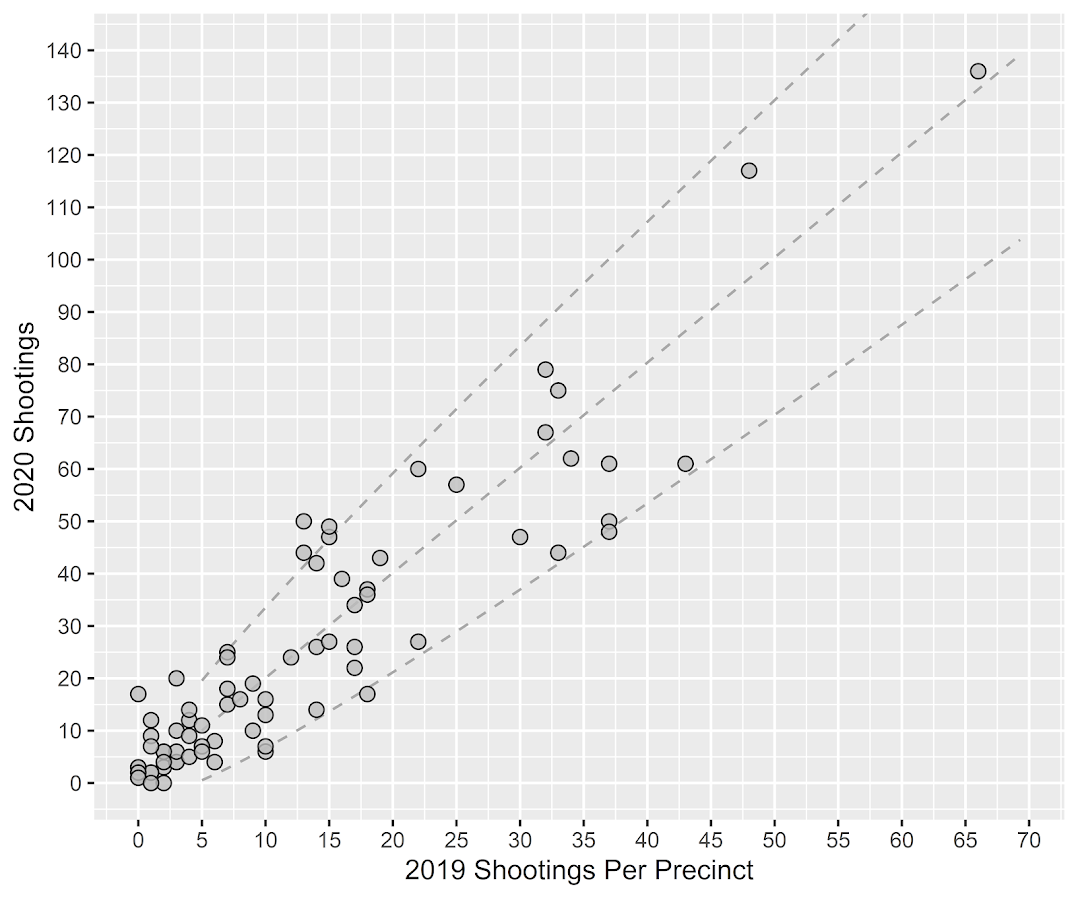The contour lines show the hypothesis that crime increased (by around 100% here). So if a point is near the middle line, it follows that doubled mark almost exactly. The upper/lower lines indicate the typical variance, which is a very good fit to the data here you can see. Very few points are outside the boundaries.

Both of these analyses point to the fact that shooting increases were widespread across NYC precincts. Pretty much everywhere doubled in the number of shootings, it is just some places had a larger baseline to double than others (and the data has some noise, you can pick out some places that did not increase if you cherry pick the data).

And as a final R note, if you want to save these graphs as a nice high resolution PNG, here is an example with Jerry’s dispersion object:

``````# Saving dispersion plot as a high res PNG
png(file = "ODI.png", bg = "transparent", height=5, width=9, units="in", res=1000, type="cairo")
output #this is the object from Jerrys crimedispersion() function earlier
dev.off()``````

Going forward I am wondering if there is a good way to do spatial monitoring for crime data like this, like some sort of control chart that takes into account both space and time. So isn’t retrospective a year later recap, but in near real time identify spatial increases.

# Other References of Interest

• Justin Nix & company have a few blog posts looking at NYC data as well. In the first they talk about the variance in cities, many are up but several are down as well in violence. A later post though updated with the clear increase in shootings in NYC.
• There are too many papers at this point for me to do a bibliography of all the Covid and crime updates, but two open examples are Matt Ashby did a paper on several US cities, and Campedelli et al have an analysis of Chicago. Each show variance again, so no universal up or down in trends, but various examples of increases or decreases both between cities and between different crime types within a city.

# A Tableau walkthough: Seasonal chart

So my workplace uses Tableau quite a bit, and I know it is becoming pretty popular for crime analysis units as well. So I was interested in trying to pick some up. It can be quite daunting though. I’ve tried to sit through a few general tutorials, but they make my head spin.

Students of mine when I teach ArcGIS have said it is so many buttons it can be overwhelming, and Tableau is much the same way. I can see the appeal of it though, in particular for analysts who exclusively use Excel. The drag/drop you can somewhat intuitively build more detailed charts that are difficult to put together in Excel. And of course out of the box it produces interactive charts you can share, which is really the kicker that differentiates Tableau from other tools.

So instead of sitting through more tutorials I figured I would just jump in and make a few interactive graphics. And along the way I will do tutorials, same as for my other crime analysis labs, for others to follow along.

And I’ve finished/posted my first tutorial, making a seasonal chart. It is too big to fit into a blog post (over 30 screenshots!). But shows how to make a monthly seasonal chart, which is a nice interactive to have for Compstat like meetings.

Here is the final interactive version, and here is a screenshot of the end result:And you can find the full walkthrough with screenshots here:

TABLEAU SEAONAL CHART TUTORIAL/WALKTHROUGH

## Some Things Crime Analysts Should Consider When Using Tableau

So first, I built this using the free version of Tableau. I don’t think the free version will cut it though for most crime analysts.

One of the big things I see Tableau as being convenient is a visualization layer on top of a database. It can connect to the live database, and so automatically update. You cannot do this though with the free version. (And likely you will need some SQL chops to get views for data in formats you can’t figure out how to coerce Tableau functions.)

So if you go through the above tutorial and say that is alot of work, well it is, but you can set it up once on a live data stream, and it just works going forward.

The licensing isn’t crazy though, and if you are doing this for data that can be shared with the public, I think that can make sense for crime analysts. For detailed report info that cannot be shared with the public, it is a bit more tricky though (and I definitely cannot help with the details for doing your own on prem server).

There are other totally free interactive dashboard like options as well, such as Shiny in R, plotly libraries (in R and python), and python has a few other interactive ones as well. The hardest part really is the server portion for any of them (making it so others can see the interactive graphic). Tableau is nice and reactive though in my experience, even when hooked up to a live data stream (but not crazy big data).

I hope to expand to my example Poisson z-score charts with error bands, and then maybe see if I can build a dashboard with some good cross-linking between panes with geo data.

For this example I am almost 100% happy with the end result. One thing I would like is for the hover behavior to select the entire line (but the tooltips still be individual months). Also would like the point at the very end to be larger, and not show the label. But these are very minor things in the end.

# My online course lab materials and musings about online teaching

I often refer folks to the courses I have placed online. Just for an update for everyone, if you look at the top of my website, I have pages for each of my courses at the header of my page. Several of these are just descriptions and syllabi, but the few lab based courses I have done over the years I have put my materials entirely online. So those are:

And each of those pages links to a GitHub page where all the lab goodies are stored.

The seminar in research focuses on popular quasi-experimental designs in CJ, and has code in R/Stata/SPSS for the weekly lessons. (Will need to update with python, I may need to write my own python margins library though!)

Grad GIS is mostly old ArcGIS tutorials (I don’t think I will update ArcPro, will see when Eric Piza’s new book comes out and just suggest that probably). Even though the screenshots are perhaps old at this point though the ideas/workflow are not. (It also has some tutorials on other open source tools, such as CrimeStat, Jerry’s Near Repeat Calculator, GeoDa, spatial regression analysis in R, and Mallesons/Andresens SPPT tool are examples I remember offhand.)

Undergrad Crime Analysis is mostly focused on number crunching relevant to crime analysts in Excel, although has a few things in Access (making SQL queries), and making a BOLO in publisher.

So for folks self-learning of course use those resources however you want. My suggestion is to skim through the syllabus, see if you want to learn about any particular lesson, and then jump right to that one. No need to slog through the whole course if you are just interested in one specific thing.

They are also freely available to any instructors who want to adapt those materials for their own courses as well.

One of the things that has disappointed me about the teaching response to Covid is instead of institutions taking the opportunity to really invest in online teaching, people are just running around with their heads cut off and offering poor last minute hybrid courses. (This is both for the kiddos as well as higher education.)

If you have ever taken a Coursera course, they are a real production! And the ones I have tried have all been really well done; nice videos, interactive quizzes with immediate feedback, etc. A professor on their own though cannot accomplish that, we would need investment from the University in filming and in scripting the webpage. But once it is finished, it can be delivered to the masses.

So instead of running courses with a tiny number of students, I think it makes more sense for Universities to actually pony up resources to help professors make professional looking online courses. Not the nonsense with a bad recorded lecture and a discussion board. It is IMO better to give someone a semester sabbatical to develop a really nice online course than make people develop them at the last minute. Once the course is set up, you really only need to administer the course, which takes much less work.

Another interested party may be professional organizations. For example, the American Society of Criminology could make an ad-hoc committee to develop a model curriculum for an intro criminology course. You can see in my course pages I taught this at one point – there is no real reason why every criminology teacher needs to strike out on their own. This is both more work for the individual teacher, as well as introduces quite a bit of variation in the content that crim/cj students receive.

Even if ASC started smaller, say promoting individual lessons, that would be lovely. Part of the difficulty in teaching a broad course like Intro to Criminology is that I am not an expert on all of criminology. So for example if someone made a lesson plan/video for bio-social criminology, I would be more apt to use that. Think instead of a single textbook, leveraging multi-media.

It is a bit ironic, but one of the reasons I was hired at HMS was to internally deliver data science training. So even though I am in the private sector I am still teaching!

Like I said previously, you are on your own for developing teaching content at the University. There is very little oversight. I imagine many professors will cringe at my description, but one of the things I like at HMS is the collaboration in developing materials. So I initially sat down with my supervisor and project manager to develop the overall curricula. Then for individual lessons I submit my slides/lab portion to my supervisor to get feedback, and also do a dry run in front of one of my peers on our data science team to get feedback. Then in the end I do a recorded lecture – we limit to something like 30 people on WebEx so it is not lagging, but ultimately everyone in the org can access the video recording at a later date.

So again I think this is a better approach. It takes more time, and I only do one lecture at a time (so take a month or two to develop one lecture). But I think that in the end this will be a better long term investment than the typical way Uni’s deliver courses.

# Checking a Poisson distribution fit: An example with officer involved shooting deaths WaPo data (R functions)

So besides code on my GitHub page, I have a list of various statistic functions I’ve scripted on the blog over the years on my code snippets page. One of those functions I will illustrate today is some R code to check the fit of the Poisson distribution. Many of my crime analysis examples rely on crime data being approximately Poisson distributed. Additionally it is relevant in regression model building, e.g. should I use a Poisson GLM or do I need to use some type of zero-inflated model?

Here is a brief example to show how my R code works. You can source it directly from my dropbox page. Then I generated 10k simulated rows of Poisson data with a mean of 0.2. So I see many people in CJ make the mistake that, OK my data has 85% zeroes, I need to use some sort of zero-inflated model. If you are working with very small spatial/temporal units of analysis and/or rare crimes, it may be the mean of the distribution is quite low, and so the Poisson distribution is actually quite close.

``````# My check Poisson function
source('https://dl.dropboxusercontent.com/s/yj7yc07s5fgkirz/CheckPoisson.R?dl=0')

# Example with simulated data
set.seed(10)
lambda <- 0.2
x <- rpois(10000,lambda)
CheckPoisson(x,0,max(x),mean(x))``````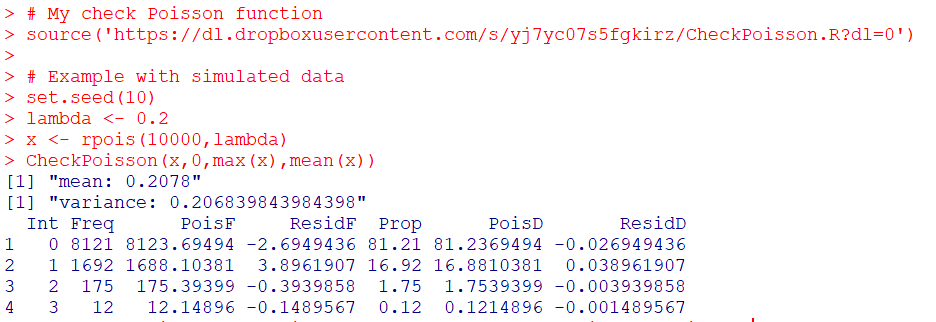Here you can see in the generated table from my `CheckPoisson` function, that with a mean of 0.2, we expect around 81.2% zeroes in the data. And since we simulated the data according to the Poisson distribution, that is what we get. The table shows that out of the 10k simulation rows, 8121 were 0’s, 1692 rows were 1’s etc.

In real life data never exactly conform to hypothetical distributions. But we often want to see how close they are to the hypothetical before building predictive models. A real life example as close to Poisson distributed data as I have ever seen is the Washington Post Fatal Use of Force data. Every year WaPo has been collating the data, the total number of Fatal uses of Police Force in the US have been very close to 1000 events per year. And even in all the turmoil this past year, that is still the case.

``````# Washington Post Officer Involved Shooting Deaths Data
oid <- read.csv('https://raw.githubusercontent.com/washingtonpost/data-police-shootings/master/fatal-police-shootings-data.csv',
stringsAsFactors = F)

# Year Stats
oid\$year <- as.integer(substr(oid\$date,1,4))
year_stats <- table(oid\$year)[1:6]
year_stats
mean(year_stats)
var(year_stats)``````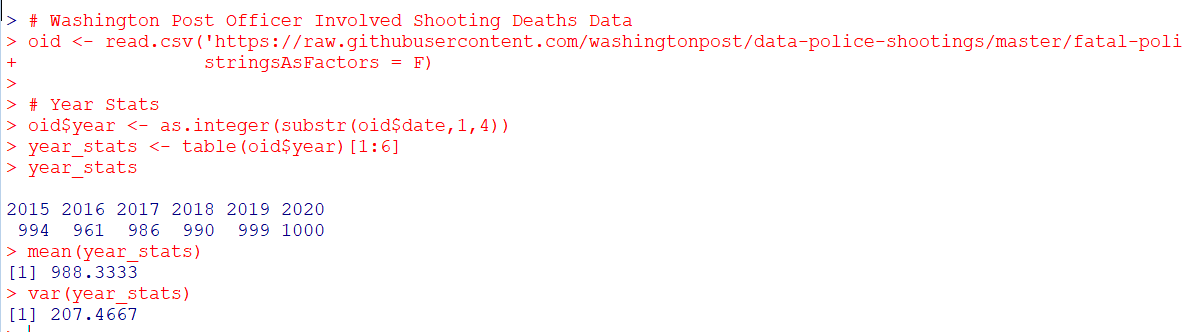One way to check the Poison distribution is that the mean and the variance should be close, and here at the yearly level the data have some evidence of underdispersion according to the Poisson distribution (most crime data is overdispersed – the variance is much greater than the mean). If the actual mean is around 990, you would expect typical variations of say around plus/minus 60 per year (`~ 2*sqrt(990)`). But that only gives us a few observations to check (6 years). We can dis-aggregate the data to smaller intervals and check the Poisson assumption. Here I aggregate to days (note that this includes zero days in the table levels calculation). Then we again check the fit of the Poisson distribution.

``````#Now aggregating to count per day
oid\$date_val <- as.Date(oid\$date)
date_range <- paste0(seq(as.Date('2015-01-01'),max(oid\$date_val),by='days'))
day_counts <- as.data.frame(table(factor(oid\$date,levels=date_range)))
head(day_counts)
pfit <- CheckPoisson(day_counts\$Freq, 0, 10, mean(day_counts\$Freq))
pfit``````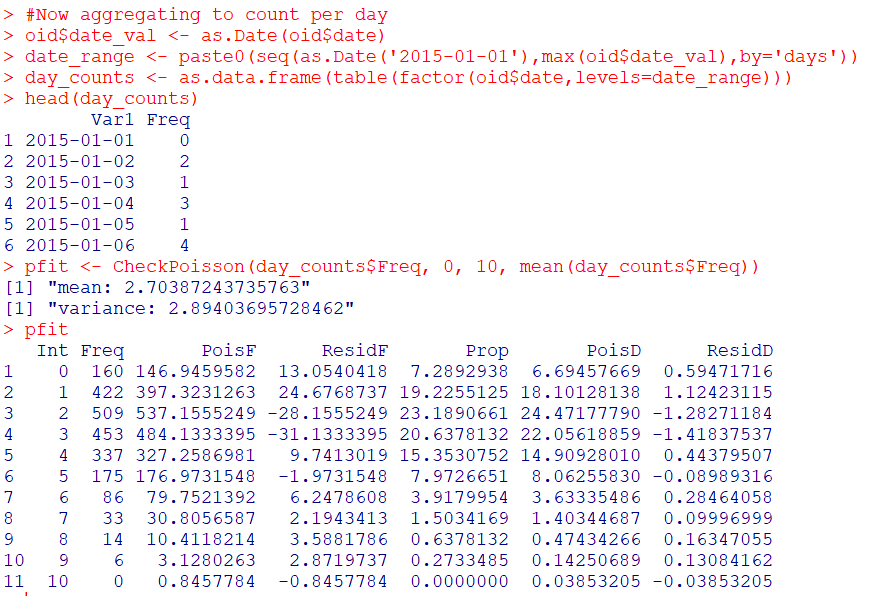According to the mean and the variance, it appears the distribution is a very close fit to the Poisson. We can see in this data we expected to have around 147 days with 0 fatal encounters, and in reality there were 160. I like seeing the overall counts, but another way is via the proportions in the final three columns of the table. You can see for all of the integers, we are less than 2 percentage points off for any particular integer count. E.g. we expect the distribution to have 3 fatal uses of force on about 22% of the days, but in the observed distribution days with 3 events only happened around 21% of the days (or `20.6378132` without rounding). So overall these fatal use of force data of course are not exactly Poisson distributed, but they are quite close.

So the Poisson distribution is motivated via a process in which the inter-arrival dates of events being counted are independent. Or in more simple terms one event does not cause a future event to come faster or slower. So offhand if you had a hypothesis that publicizing officer fatalities made future officers more hesitant to use deadly force, this is not supported in this data. Given that this is officer involved fatal encounters in the entire US, it is consistent with the data generating process that a fatal encounter in one jurisdiction has little to do with fatal encounters in other jurisdictions.

(Crime data we are often interested in the opposite self-exciting hypothesis, that one event causes another to happen in the near future. Self-excitation would cause an increase in the variance, so the opposite process would result in a reduced variance of the counts. E.g. if you have something that occurs at a regular monthly interval, the counts of that event will be underdispersed according to a Poisson process.)

So the above examples just checked a univariate data source for whether the Poisson distribution was a decent fit. Oftentimes academics are interested in whether the conditional distribution is a good fit post some regression model. So even if the marginal distribution is not Poisson, it may be you can still use a Poisson GLM, generate good predictions, and the conditional model is a good fit for the Poisson distribution. (That being said, you model has to do more work the further away it is from the hypothetical distribution, so if the marginal is very clearly off from Poisson a Poisson GLM probably won’t fit very well.)

My `CheckPoisson` function allows you to check the fit of a Poisson GLM by piping in varying predicted values over the sample instead of just one. Here is an example where I use a Poisson GLM to generate estimates conditional on the day of the week (just for illustration, I don’t have any obvious reason fatal encounters would occur more or less often during particular days of the week).

``````#Do example for the day of the week
day_counts\$wd <- weekdays(as.Date(day_counts\$Var1))
mod <- glm(Freq ~ as.factor(wd) - 1, family="poisson", data=day_counts)
#summary(mod), Tue/Wed/Thu a bit higher
lin_pred <- exp(predict(mod))
pfit_wd <- CheckPoisson(day_counts\$Freq, 0, 10, lin_pred)
pfit_wd``````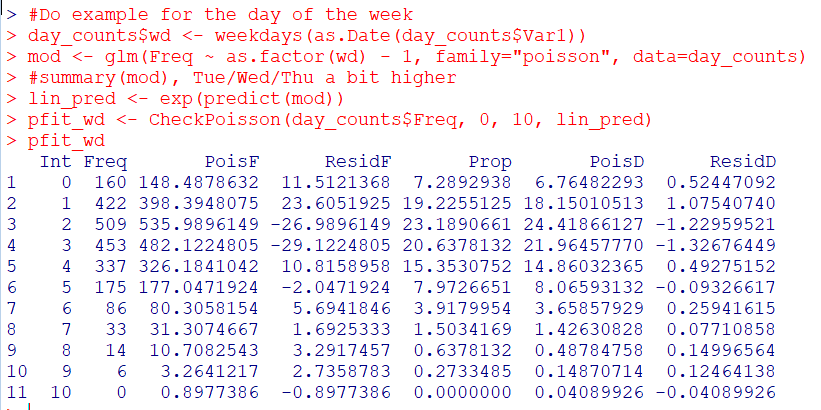You can see that the fit is almost exactly the same as before with the univariate data, so the differences in days of the week does not explain most of the divergence from the hypothetical Poisson distribution, but again this data is already quite close to a Poisson distribution.

So it is common for people to do tests for goodness-of-fit using these tables. I don’t really recommend it – just look at the table and see if it is close. Departures from hypothetical can inform modeling decisions, e.g. if you do have more zeroes than expected than you may need a negative binomial model or a zero-inflated model. If the departures are not dramatic, variance estimates from the Poisson assumption are not likely to be dramatically off-the-mark.

But if you must, here is an example of generating a Chi-Square goodness-of-fit test with the example Poisson fit table.

``````# If you really want to do a test of fit
chi_stat <- sum((pfit\$Freq - pfit\$PoisF)^2/pfit\$PoisF)
df <- length(pfit\$Freq) - 2
dchisq(chi_stat, df)``````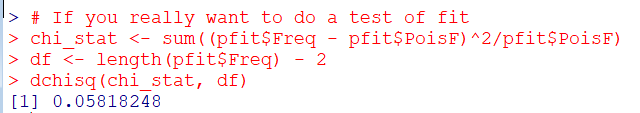So you can see in this example the p-value is just under 0.06.

I really don’t recommend this though for two reasons. One is that with null hypothesis significance testing you are really put in a position that large data samples always reject the null, even if the departures are trivial in terms of the assumptions you are making for whatever subsequent model. The flipside of this is that with small samples the test is underpowered, so there are never many good scenarios where it is useful in practice. Two, you can generate superfluous categories (or collapse particular categories) in the Chi-Square test to increase the degrees of freedom and change the p-value.

One of the things though that this is useful for is checking the opposite, people fudging data. If you have data too close to the hypothetical distribution (so very high p-values here), it can be evidence that someone manipulated the data (because real data is never that close to hypothetical distributions). A famous example of this type of test is whether Mendel manipulated his data.

I intentionally chose the WaPo data as it is one of the few that out of the box really appears to be close to Poisson distributed in the wild. One of my next tasks though is to do some similar code for negative binomial fits. Like Paul Allison, for crime count data I rarely see much need for zero-inflated models. But while I was working on that I noticed that the parameters in NB fits with even samples of 1,000 to 10,000 observations were not very good. So I will need to dig into that more as well.

# The WDD test with different pre/post time periods

Eric Piza asked the other day if my and Jerry’s WDD test can be used when the pre/post time periods are different. The answer is yes out of the box, the identification strategy does not rely on equality of time periods. So for example, say we had two years pre and one year post data, and the crime counts in treated/control looked like this:

``````         Pre  Post
Treated   80    20
Control  100    50``````

So then our difference-in-difference Poisson estimate of the treatment effect would be:

``(20 - 80) - (50 - 100) =  -10``

What the parallel trends assumption means here is that since you saw a decrease in 50 crimes in the control area, you would expect a decrease of 50 crimes in the treated area as well. The variance of this estimate is then `20 + 80 + 50 + 100 = 250`, and so the standard error is `sqrt(250) ~ 15.8`. So this is not a statistically significant effect.

It is hard to interpret this effect size though, since it is not a standard unit of time comparison. Also the variance of the estimate will be larger if you have a longer pre time period, which is the opposite of what you want. We can actually amend the statistic though to be a per-unit-time comparison, which will reduce the variance of the estimate. It ends up being similar to my prior post on adding Harm Weights to the WDD, you can’t just pipe in the per unit time estimates in the spreadsheet I shared, but I will show here how to incorporate them into the estimator (and share some python code to show the estimator behaves as expected in simulations).

So again with a pre-time period of 2 years, and post of 1 year, we could do the prior table as per year estimates.

``````         Pre  Post
Treated   40    20
Control   50    50``````

And here our estimate of the crime reduction effect is different:

``(20 - 40) - (50 - 50) =  -20``

So with a Poisson variable with a mean of 100, the variance of that variable is also 100. So here we are dividing that 100 by a constant 2 – this changes the variance to 100/(2^2). (`Var(X*a) = a^2*Var(X)` where `X` is a random variable and `a` is a constant.) The post variables are simply divided by one, so does not change their variance. So to carry this forward to our standard error estimate, we would calculate:

``20/1 + 40/4 + 50/1 + 50/4 = 92.5``

So you can see that our variance estimate here is much smaller, and that the standard error is `sqrt(92.5) ~ 9.6`. So here the reduction is right on the border of a statistically significant reduction in crimes. A 95% confidence interval would be `-20 +/- 2*9.6 ~ [-1, -39]`. Here the WDD estimate is easier to interpret as well, and that confidence interval corresponds to a per year estimate reduction of somewhere between 1 and 39 crimes.

Below I share some python code to conduct simulations similar to the original WDD paper. This code will then establish the estimator has the null distribution as expected (when there are no changes it really is a standard normal distribution) and that the confidence intervals have coverage like you would expect.

# Python Simulation Code

For set up, I import the libraries I need (stat distributions, numpy and pandas). I am not going to go into detail into the functions, but it allows you to generate simulated distributions in various ways to conduct analysis of the properties of my time weighted estimator I have specified above.

``````'''
WDD Simulation with differing time periods
Andy Wheeler
'''

import pandas as pd
import numpy as np
from scipy.stats import norm
from scipy.stats import poisson
from scipy.stats import uniform

#This works for the scipy functions
np.random.seed(seed=10)

# A function to generate the WDD estimate for simulated data
def wdd_sim(treat0,treat1,cont0,cont1,pre,post):
tr_cr_0 = poisson.rvs(mu = treat0, size=int(pre)).sum()
co_cr_0 = poisson.rvs(mu = cont0, size=int(pre)).sum()
tr_cr_1 = poisson.rvs(mu = treat1, size=int(post)).sum()
co_cr_1 = poisson.rvs(mu = cont1, size=int(post)).sum()
est = ( tr_cr_1/post - tr_cr_0/pre ) - ( co_cr_1/post - co_cr_0/pre )
post2 = (1/post)**2
pre2 = (1/pre)**2
var_est = tr_cr_0*pre2 + tr_cr_1*post2 + co_cr_0*pre2 + co_cr_1*post2
true_val = ( treat1 - treat0 ) - ( cont1 - cont0 )
z_score = est / np.sqrt(var_est)
return (est, var_est, true_val, z_score)

def make_data(n, treat0, treat1, cont0, cont1, pre, post):
base = pd.DataFrame( range(n), columns=['index'])
base['treat0'] = treat0
if treat1 is not None:
base['treat1'] = treat1
else:
base['treat1'] = base['treat0']
if cont0 is not None:
base['cont0'] = cont0
else:
base['cont0'] = base['treat0']
if cont1 is not None:
base['cont1'] = cont1
else:
base['cont1'] = base['cont0']
base.drop(columns='index',inplace=True)
base['pre'] = pre
base['post'] = post
sim_vals = base.apply(lambda x: wdd_sim(**x), axis=1, result_type='expand')
sim_vals.columns = ['est','var_est','true_val','z_score']
return pd.concat([base,sim_vals], axis=1)``````

So for a first example, this code generates treatment/control areas with a Poisson mean of 5 in both the pre/post time periods. But, the pre time period is 4 units of time, and the post time period is only 1 unit. So this means there is no change, and the Z score estimator should on average have a 0 estimate and a standard deviation of 1. I do 10,000 simulations to keep it going a bit faster, but you can up that if you want.

``````# No change, with baseline of 5 crimes per unit time
sim_dat = make_data(10000, 5, 5, 5, 5, 4, 1)
sim_dat['z_score'].describe()``````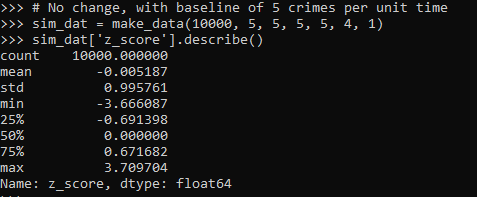So here we can see these 10k simulated Poisson data have a mean z-score of 0 and a standard deviation of 1, right like we expected.

So I haven’t extensively tested, but if you have average crime counts well under 5, I would be a bit hesitant to use this estimator. (So you either need larger area aggregations or larger time aggregations.) Although you could do simulations on your own to see how it holds up.

The way I wrote the functions you can also pass in random variables as well, so here is an example with again no change, but the baseline varies uniformily from 5 to 100. And here also the pre time periods are 6, and the post time period is again just 1.

``````# Can pass in random functions instead of constant values
sim_n = 10000
tf = uniform.rvs(loc=5, scale=100, size=sim_n)

sim_dat2 = make_data(sim_n, tf, None, None, None, 6, 1)
sim_dat2.head()
sim_dat2['z_score'].describe()``````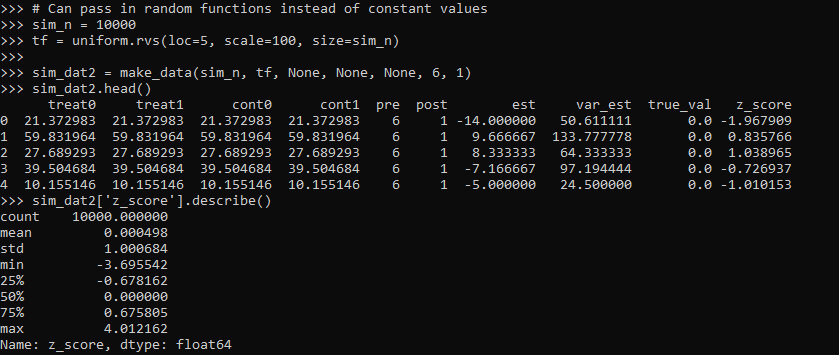So you can see the base simulated dataset pre/post always has the same means, but instead of being a set of constant 5’s, it changes for each row (simulation) in the dataset. And again the null distribution is right on the money with a mean of 0 and standard deviation of 1.

So those are examples of the null distribution of no changes in the time weighted estimator. This establishes that the false positive alpha rates are as you would expect. E.g. if you use the usual p-value < 0.05, if the differences are really 0 you only have a false positive reject the null 5 times out of 100.

But we also want to establish that when there is a difference, the estimator is not biased and that the variance estimates are correct. For the later part looking at the coverage rates of the confidence intervals is one way to do that. So here I show that with my hypothetical example in the intro part of this blog, the 95% and 90% confidence interval coverage rates are exactly as they should be. And the z-score estimate is right about where it should be as well.

``````# Lets look at the coverage rate for a decline from 40 to 20
def cover(data, ci=0.95):
mult = (1 - ci)/2
nv = norm.ppf(1 - mult)
dif = nv*np.sqrt( data['var_est'] )
low = data['est'] - dif
high = data['est'] + dif
cover = ( data['true_val'] > low) & ( data['true_val'] < high )
return cover

sim_dat3 = make_data(sim_n, 40, 20, 50, 50, 2, 1)
sim_dat3.head()

# This should be centered on 2
sim_dat3['z_score'].describe()

# Should be ~ 0.9
co_90 = cover(sim_dat3, ci=0.9)
co_90.mean()

# Should be ~ 0.95
co_95 = cover(sim_dat3, ci=0.95)
co_95.mean()``````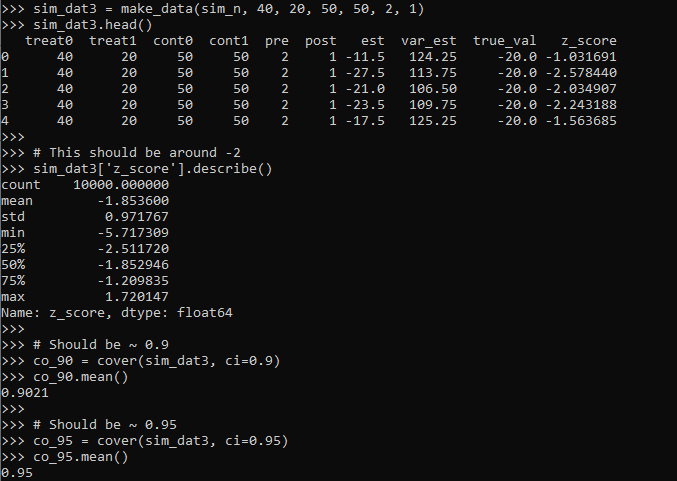So you can see the coverage is right on the money. The estimator is slightly biased downward in this simulation (should get a z-score on average around -2, but here the mean is -1.85). But it is good enough IMO to not worry about much in this situation.

Again, the original estimator without weighted for time is fine, if we do the same motions without doing weighting for different time periods, the coverage is still all fine and dandy.

``````# Note you can do the same coverage estimate without time weighted
sim_dat4 = make_data(sim_n, 80, 20, 100, 50, 1, 1)
sim_dat4.head()

# This should be around -0.6
sim_dat4['z_score'].describe()

co_90w = cover(sim_dat4, ci=0.9)
co_90w.mean()

co_95w = cover(sim_dat4, ci=0.95)
co_95w.mean()``````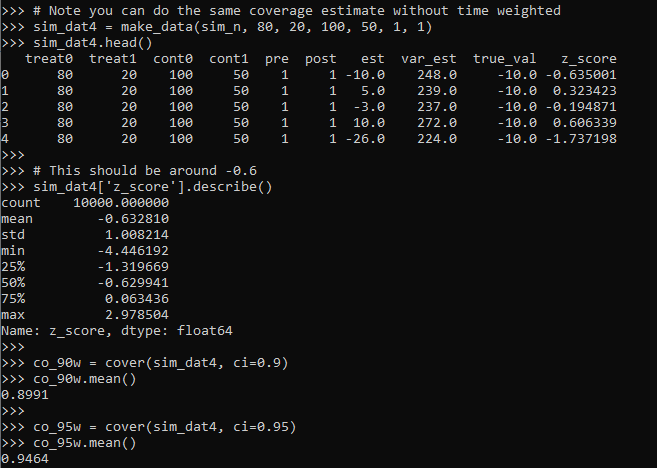So you can see again coverage is right on the money, and the z-score estimator actually has less bias than the time weighted one, it is right on the money as expected.

So why would you prefer the time weighted estimator if it shows more bias? It is because it has a lower variance, this code shows the length of the confidence intervals in the simulations.

``````# Does it make a difference?
def len_ci(data, ci=0.95):
mult = (1 - ci)/2
nv = norm.ppf(1 - mult)
dif = nv*np.sqrt( data['var_est'] )
low = data['est'] - dif
high = data['est'] + dif
return high - low

len4 = len_ci(sim_dat4)
len4.describe()

len3 = len_ci(sim_dat3)
len3.describe()``````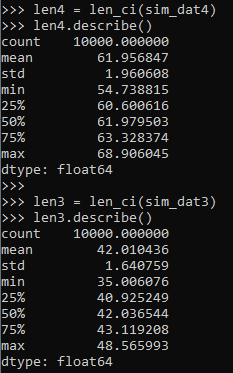So you can see here that the non-time weighted estimator tends to have a confidence interval with a length of 62, whereas the time weighted estimator has a confidence interval on average of 42.

So above establishes that the time weighted estimator behaves as you would expect. You can also use this code to conduct some potential power analyses. So for the time weighted estimator we show, even though the reduction is around 50% in the treated area (going from 40 to 20), the power is not great, around 60%.

``````# Example power analyses, ONE TAILED
def reject_rate(data, alpha=0.05):
p_vals = norm.cdf(data['z_score'])
return p_vals < alpha

r3 = reject_rate(sim_dat3)
r3.mean()``````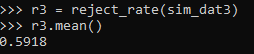So this means if you did this experiment in real life and it was that effective, you would still fail to reject the null of no differences 2/5 times.

But what if we say we will get more historical data? So 4 years back instead of just 2? How does that impact our power estimates?

``````# How about with more historical data
sim_dat5 = make_data(sim_n, 40, 20, 50, 50, 4, 1)
r5 = reject_rate(sim_dat5)
r5.mean()``````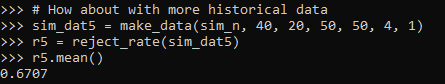The power goes up by alittle, to 0.67. The same is true if we up the post period to 4 time periods instead of 1:

``````# How about with more post data
sim_dat6 = make_data(sim_n, 40, 20, 50, 50, 4, 4)
r6 = reject_rate(sim_dat6)
r6.mean()``````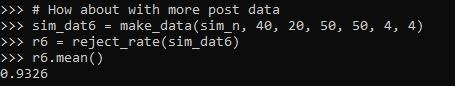So now in this example you have an over 90% power to detect a crime reduction, going from 40 to 20 per time period (where the control has an average of 50 crimes per time period), if you have 4 pre time periods and 4 post time periods.

# Future Stuff

So a few caveats with this. For one, you may think that since dividing per time period reduces the variance, why not divide by smaller time slivers. So instead of one year, why not divide by 365 days?

I have not studied extensively this property of the estimator. So I cannot say how it behaves with more/less time aggregation into smaller Poisson estimates. You will need to take that on yourself if you want to examine very fine time units and very small Poisson counts per unit time. Again I think a baseline rule of thumb that they should not be lower the 5 counts per unit time is the best advice I can give without doing simulations for your exact circumstances.

A second part is that with longer time periods comes the risk that the control areas are not as good. This is a problem intrinsic to synthetic control analysis as well (that I don’t believe anyone has a particular answer to). And I don’t have an answer either.

For the pre-time period, you can check the parallel trends assumption by simply plotting the two time series, they should be close to in step with one another. So that is not a big deal. But with the post time period, I think if you monitor long enough they will eventually depart from one another.

So I think it is best to set up a time period at the start you have committed to doing the experiment. And you can use the power analysis simulations like I showed to help you figure out that period. But it may be possible to extend this WDD estimate to continuously monitor an intervention (see here for example).

# New book: Micro geographic analysis of Chicago homicides, 1965-2017

In joint work with Chris Herrmann and Dick Block, we now have a book out – Understanding Micro-Place Homicide Patterns in Chicago (1965 – 2017). It is a Springer Brief book, so I recommend anyone who has a journal article that is too long that this is a potential venue for the work. (Really this is like the length of three journal articles.)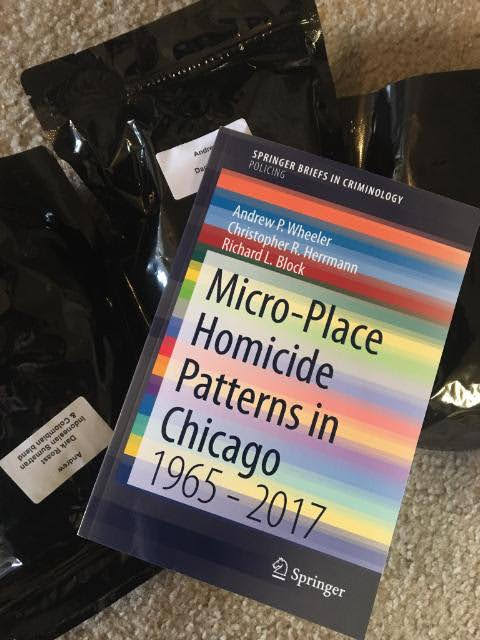A few things occurred to prompt me to look into this. First, Chicago increased a big spike of homicides in 2016 and 2017. Here is a graph breaking them down between domestic related homicides and all other homicides. You can see all of the volatility is related to non-domestic homicides.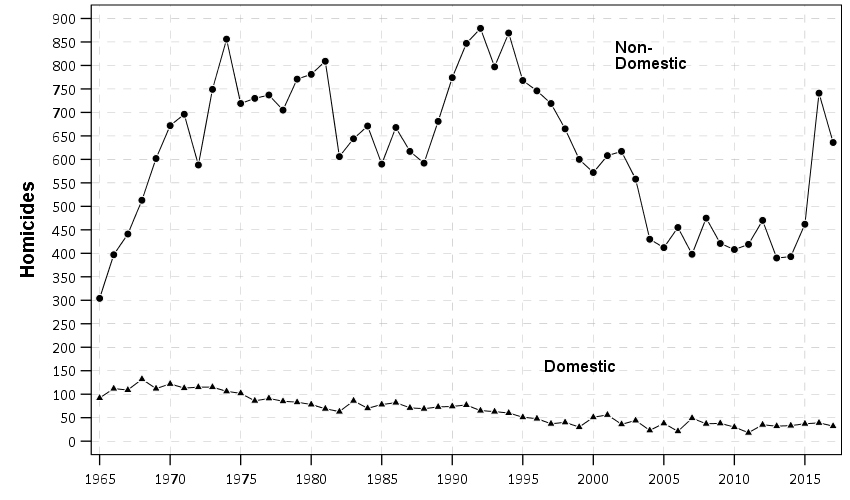So this (at least to me) begs the question of whether those spiked homicides show similar characteristics compared to historical homicides. Here we focus on long term spatial patterns and micro place grid cells in the city, 150 by 150 meter cells. Dick & Carolyn Block had collated data, including the address where the body was discovered, using detective case notes starting in 1965 (ending in 2000). The data from 2000 through 2017 is the public incident report data released by Chicago PD online. Although Dick and Carolyn’s public dataset is likely well known at this point, Dick has more detailed data than is released publicly on ICPSR and a few more years (through 2000). Here is a map showing those homicide patterns aggregated over the entire long time period.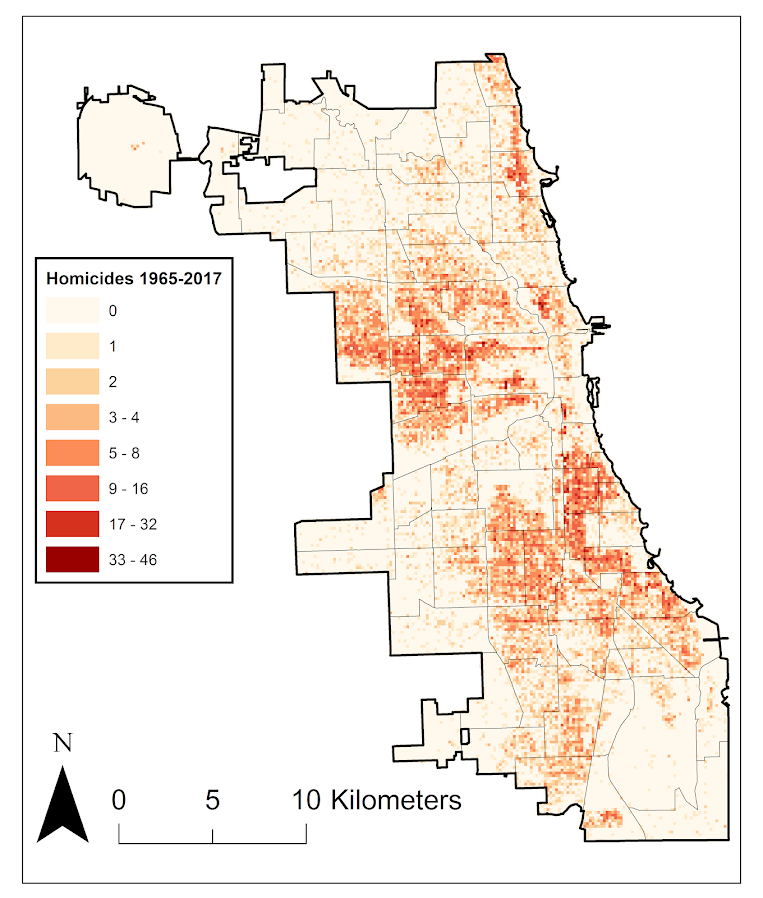So we really have two different broad exploratory analyses we employed in the work. One was to examine homicide clustering, and the other was to examine temporal patterns in homicides. For clustering, we go through a ton of different metrics common in the field, and I introduce even one more, Theil’s decomposition for within/between neighborhood clustering. This shows Theil’s clustering metric within neighborhoods in Chicago (based on the entire time period).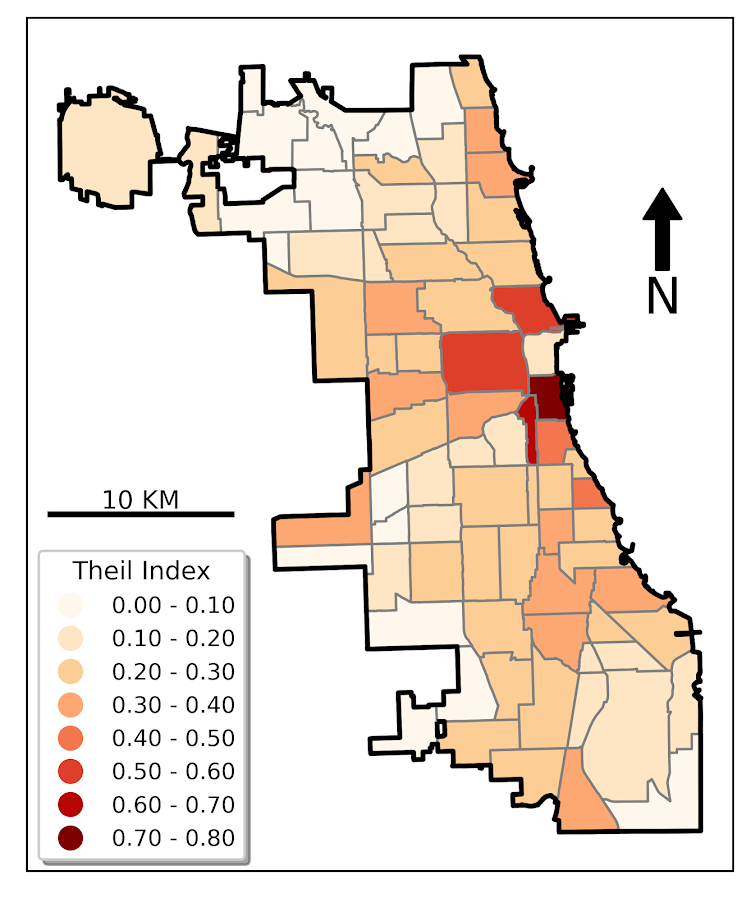So areas around the loop showed more clustering in homicides, but here it appears it is somewhat confounded with neighborhood size – smaller neighborhoods appear to have more clustering. This is sort of par for the course for these clustering metrics (we go through several different Gini variants as well), in that they are pretty fickle. You do a different temporal slice of data or treat empty grid cells differently the clustering metrics can change quite a bit.

So I personally prefer to focus on long term temporal patterns. Here I estimated group based trajectory models using zero-inflated Poisson models. And here are the predicted outputs for those grid cells over the city. You can see unlike prior work David Weisburd (Seattle), myself (Albany), or Martin Andresen (Vancouver) has done, they are much more wavy patterns. This may be due to looking over a much longer horizon than any of those prior works though have.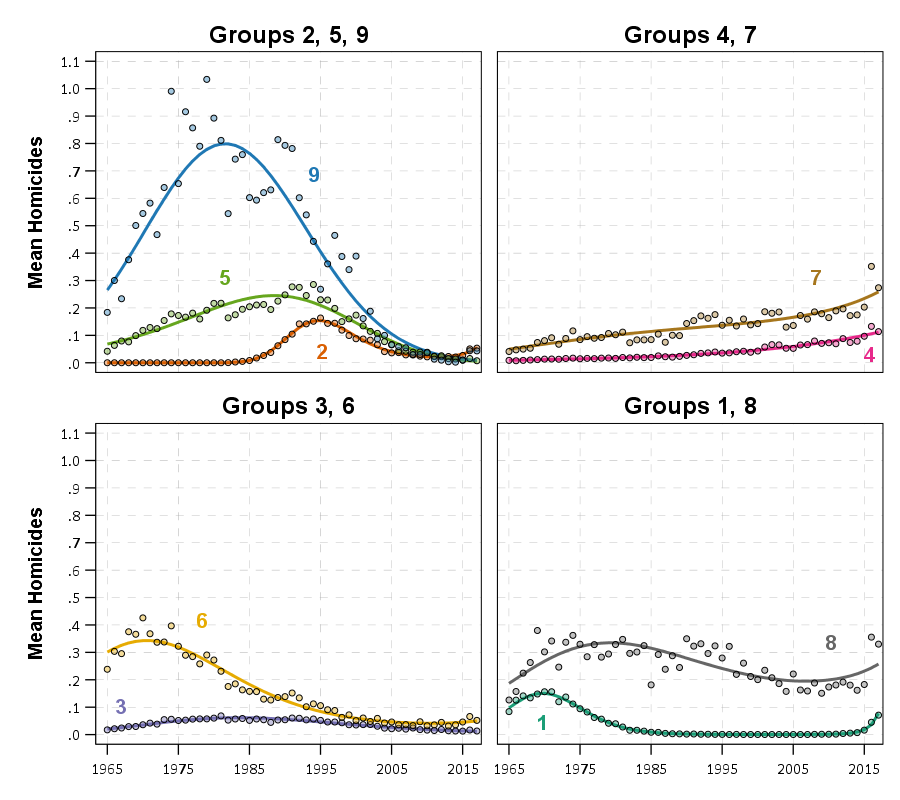The big wave, Group 9, ends up being clearly tied to former large public housing projects, which their demolitions corresponds to the downturn.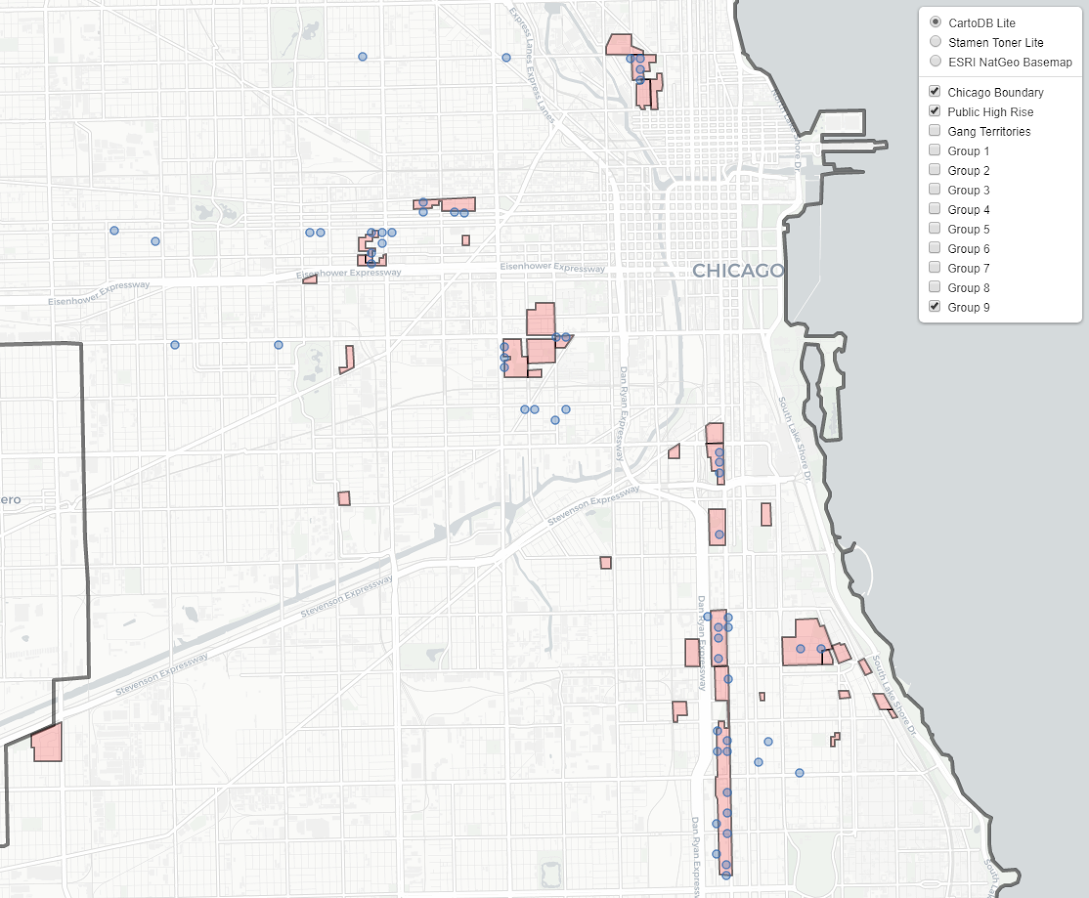I have an interactive map to explore the other trajectory groups here. Unfortunately the others don’t show as clear of patterns as Group 9, so it is difficult to answer any hard questions about the uptick in 2016/2017, you could find evidence of homicides dispersing vs homicides being in the same places but at a higher intensity if you slice the data different ways.

Unfortunately the analysis is never ending. Chicago homicides have again spiked this year, so maybe we will need to redo some analysis to see if the more current trends still hold. I think I will migrate away from the clustering metrics though (Gini and Theil), they appear to be too volatile to say much of anything over short term patterns. I think there may be other point pattern analysis that are more diagnostic to really understand emerging/changing spatial patterns.

The coffee next to the cover image is Chris Herrmann’s beans, so go get yourself some as well at Fellowship Coffee!

# Changepoints in CCTV Effects

So I am a big fan of using splines in regression equations to model non-linear effects. But a limitation of these is that you need to upfront say how many knots you want, as well as where the knots are. So I have explored a bit on fitting models that can identify the changepoints themselves. It was a tricky road, I tried building some in deep learning using pytorch, then tried variational auto-encoders in pyro, then pystan (marginalizing the changepoint out), and then pymc3 (using different samplers). All of my attempts failed! But when I used the R mcp library (Lindeløv, 2020), it was able to find my changepoint using simulated data. (It uses JAGS under the hood, no idea why JAGS behaved better than my other attempts.)

# Usecase: Dropoff effect of CCTV on clearance rates

So in spatial criminology, a popular hypothesis is estimating distance decay effects. Ratcliffe (2012) was the first example of using a changepoint regression model to do this, showing a changepoint in the effect of bars on the spatial density of crime nearby. This has been replicated in Xu & Griffiths (2017), and in my work using machine learning and partial dependence plots I show similar changepoint patterns as well (Wheeler & Steenbeek, 2020).

One example use case though I want to mention is not in terms of estimating the spatial density of crime, but with the characteristics of the crime events themselves. Sometimes people I think mistakenly think since I have spatial data, I need to aggregate it to some areal unit, and then do analysis of that areal unit data. That approach is not per-se wrong, but is sometimes a step removed from what you want, and can result in some tricky inferences.

Take for example a recent paper looking at clearances and using RTM by Kennedy et al. (2020). What they do is spatially aggregate homicides cleared and homicides not cleared, and run RTM on each. You might be tempted to interpret if a factor is selected for both models that it does not impact clearances, but it also depends on the size of the effect. So for example, in Brooklyn for drug markets they report a rate ratio of 3.1 and 2.4 (both at the same spatial distance). To translate this into a clearance rate, you need to add the two density estimates for all cases, and then take the cleared cases as the numerator.

``````# Example R code
clear <- exp(-0.1 + log(3.1))
nonclear <- exp(-0.1 + log(2.4))
prop <- clear/(clear + nonclear)
prop #0.5636364``````

Here I am treating `-0.1` as the intercept. So here this is lower, but close to the overall clearance in Brooklyn, 58%. This 56% will be the estimate iff the intercept for each equation is the same, if they are not though it could change the clearance rate estimate either way. Since the Kennedy paper did not report this, we cannot know. So for instance, if we change the intercept estimates so clearances are higher and non-clearances are lower, we get an estimate that drug markets increase clearances slightly, not decrease them:

``````clear <- exp(-0.05 + log(3.1))
nonclear <- exp(-0.2 + log(2.4))
prop <- clear/(clear + nonclear)
prop #0.6001124``````

In this example it probably won’t push them too far either way, but takes a bit of work going from the aggregate data analysis to the estimate we want, how those spatial risk factors impact the clearance rate. There is an easier way though – just incorporate your spatial features, such as the distance the nearest crime generator factor, and estimate a model on the micro level incident data. This is what Kennedy et al. (2020) do later in the paper when incorporating the RTM predictions – I just think they should have done the RTM machinery directly on this problem, instead of the two-step approach.

Examples of my work I have done this approach in the past (incorporating spatial data into the micro level incidents) is with fatalities from gun shot wounds (Circo & Wheeler, 2020). We actually investigated non-linear effects though of distance/drive-time, and did not find evidence of that. Going back to the crime clearance example though, another pre-print I examine the effects of CCTV cameras and find a diminishing effect of case clearances given the distance to the camera (Jung & Wheeler, 2019).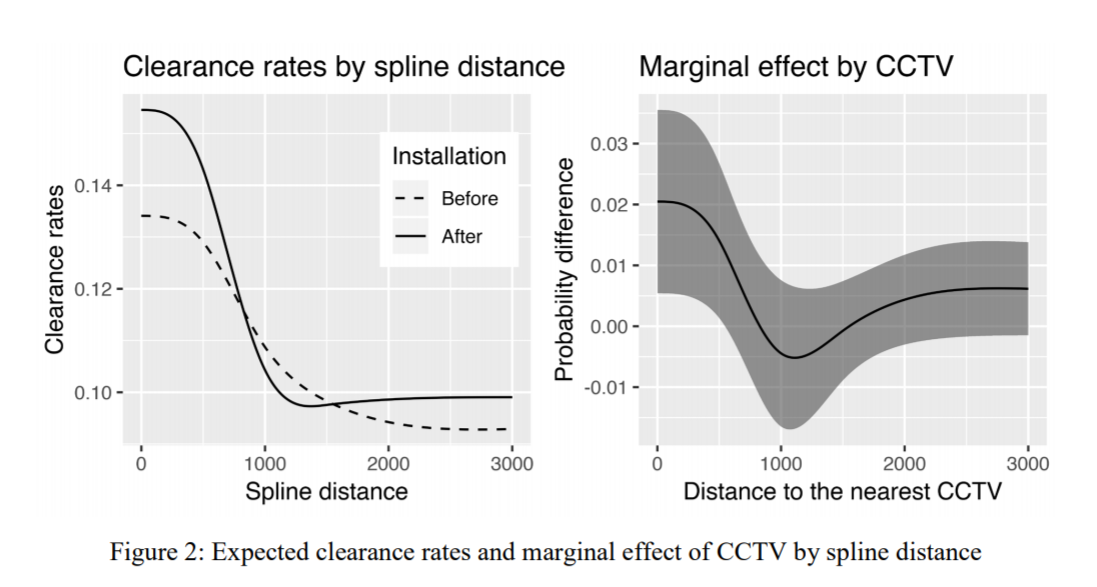So here we use a pre-post design to show there are some selection effects, and we do further analysis to show this camera bump in clearances is only limited to thefts. But we set the splines at 500, 1000, and 1500 feet pre-emptively for the analysis. A reviewer critique of this is that those three locations are arbitrary (which is correct), so here I will see if I do a changepoint model that allows us to find the knot locations if it will show the same ones.

The idea behind this analysis is that CCTV are often used in investigations. Yeondae is an officer in Korea, same as here in the states first things detectives do is to go and grab CCTV footage. Analysis of cameras are often aggregated to their viewsheds, but I think estimating distance decay effects make as much sense. So events closer to the cameras presumably will provide more clear evidence than events at the border of the viewshed. A second point is that even if the event takes place off-camera, there may be evidence cross by the camera viewshed. Detectives will often try to follow individuals across multiple cameras. So both of those factors suggest a distance decay effect both within a cameras viewshed and a decaying effect even outside of the viewshed. (In addition to this, geo coordinates of crime locations are not perfectly accurate measures either, so that could cause effects outside of the viewshed as well.)

Here I am just limiting the data to the post camera data within 3000 feet for thefts, which still is over 26,000 observations. I’ve posted the data/code to follow along here.

# Analysis using mcp in R

Again given my hardship in coding this up myself in python, I created a simulated data example and checked the results using mcp (which you can check in my code). Since mcp recovered my simulated changepoint, (and my python attempts did not), going to go ahead with the mcp library! First, we will import my clearance data and get rid of a few missing cases.

``````#################
library(mcp)
library(ggplot2)
set.seed(10)
#can see I planned on doing this in pytorch at first!
setwd('D:\\Dropbox\\Dropbox\\Documents\\BLOG\\changepoint_pytorch\\Analysis')
theft_clear <- read.csv('PostTheft_CCTV.csv')
theft_clear <- theft_clear[complete.cases(theft_clear),]
#################``````

So first for a reference, if I assume there is a linear changepoint at 1000 feet, here are what my results look like. Note here that this is not aggregated data to spatial locations, each row in this dataset is a theft offense, whether it was cleared, and the distance to the nearest CCTV camera.

``````#################
#What are the coefficients if assume a changepoint of 1000 feet
theft_clear\$x_dif <- (theft_clear\$CAM.DIST - 1000)*(theft_clear\$CAM.DIST > 1000)
theft_mod <- glm(formula = 'STATUSi ~ CAM.DIST + x_dif', family = "binomial", data = theft_clear)
summary(theft_mod) #This gives an estimate of
#################``````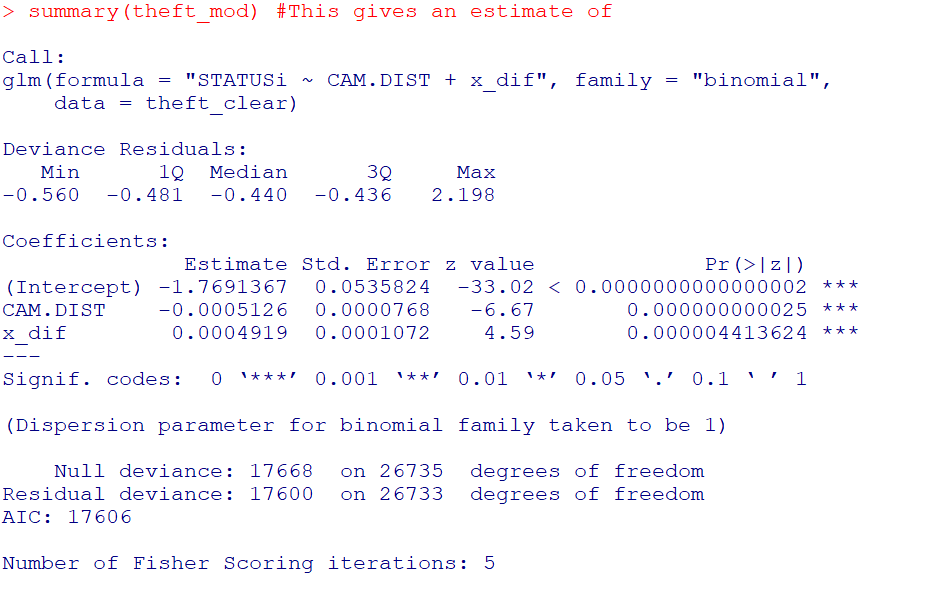And here you can visualize the results alittle easier than trying to back out probabilities for the regression equation:

``````#################
pred_mod <- predict(theft_mod,type='response')
plot(theft_clear\$CAM.DIST,pred_mod, main="Changepoint at 1000 ft",
xlab="Distance from Camera (ft)", ylab="Probability Clearance")
#################``````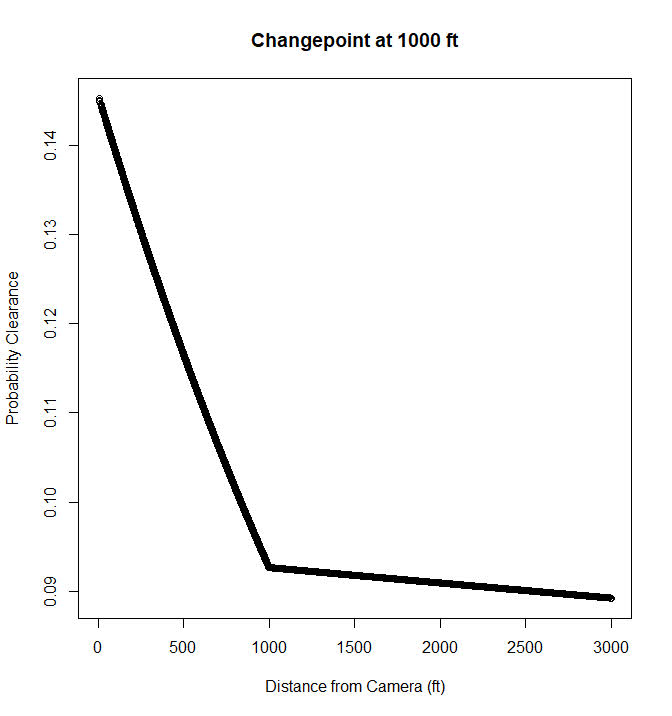So this shows clearances nearby cameras in Dallas are around 15%, and they trail off to around 9% at 1000 feet. After that they continue to tail off, but are nearly flat. But again that is assuming a change point at 1000 feet. But the mcp package lets us actual estimate the changepoint itself using Bayesian regression. Here is the set up that is equivalent to my formulation earlier, in that the changepoint cannot be discontinuous.

``````#################
theft_clear\$x <- theft_clear\$CAM.DIST
model = list(
STATUSi | trials(const) ~ 1 + x,
~ 0 + x  #joined changing rate
)

fit = mcp(model, data = theft_clear, family = binomial(), iter = 3000, adapt = 500)
#################``````

And then if you are following along you can go ahead and take a nap (maybe took 2 hours on my machine?), and when we get back `summary(fit)` gives us: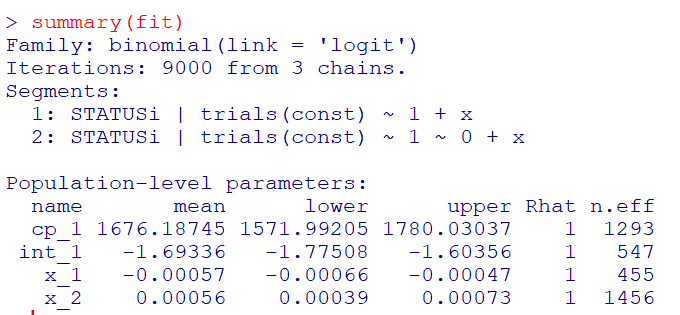So we have very similar coefficients to the manual changepoint model earlier, but the changepoint is around 1600 feet, not 1000. (Although note these are Bayesian credible intervals, not frequentist confidence intervals.) And now to make a nice plot of the fitted model.

``````#Fitted values for new data
newdat <- data.frame(x = (0:300)*10)
newdat\$const <- 1
newdat\$CAM.DIST <- newdat\$x
res <- fitted(fit, newdata = newdat)

p_pred <- ggplot(data=res) +
geom_line(size=1.2, color='black', aes(x = x, y = fitted)) +
geom_ribbon(alpha=0.5, fill='black', aes(x = x, ymin=Q2.5 , ymax=Q97.5)) +
scale_x_continuous(name="Feet from Camera",breaks=seq(0,3000,500),minor_breaks=NULL) +
scale_y_continuous(name="P(Clearance)",breaks=seq(0.06,0.16,0.02),minor_breaks=NULL) +
theme_bw() + theme(panel.grid.major = element_line(colour = 'grey', linetype = 'dashed', size=0.1)) +
theme(text = element_text(size=20))

p_pred``````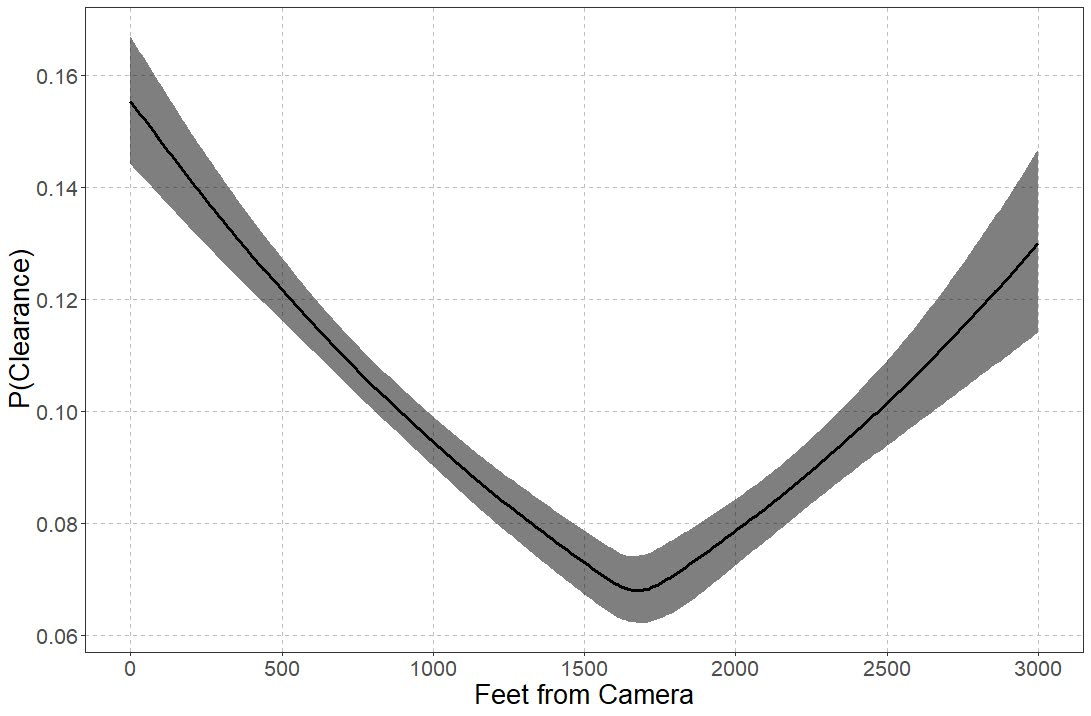So you can see that here it is a nearly linear drop off until 1600 feet, and then starts to climb back up. The climb up I think is likely due to selection effects, but we can’t 100% rule out displacement effects. Displacement effects could occur with cameras if detectives prioritize events around cameras and de-prioritize other events not nearby cameras. Skeptical that applies to thefts in Dallas though, as they very rarely will be assigned a detective at all.

# Wrap Up

So this ended up taking me for a few different turns. One of the things I wanted to be able to test multiple changepoints, maybe if I can ever get pymc3 to give me a reasonable fit, this example is a good illustration. That should also maybe say if you should have no changepoint as well. I think maybe it is much harder to fit those models with binomial data though than with continuous (maybe good for another blog post as well, did simulations at first with 1000 observations and that was a bad idea).

One thing that would be good for evaluating whether change points are reasonable are out of sample predictive comparisons. So say estimate a no changepoint model, a linear changepoint model, and then a model with fixed spline locations. Then see which of those better fits the out of sample data. But since this is a blog post, will leave it as is. But this is a simple illustration to extend prior spatial analysis of changepoints in distance decay effects to one example – crime clearances and CCTV cameras – that I think makes alot of sense.

# Amending the WDD test to incorporate Harm Weights

So I received a question the other day about amending my and Jerry Ratcliffe’s Weighted Displacement Difference (WDD) test to incorporate crime harms (Wheeler & Ratcliffe, 2018). This is a great idea, but unfortunately it takes a small bit of extra work compared to the original (from the analysts perspective). I cannot make it as simple as just piping in the pre-post crime weights into that previous spreadsheet I shared. The reason is a reduction of 10 crimes with a weight of 10 has a different variance than a reduction of 25 crimes with a weight of 4, even though both have the same total crime harm reduction (10*10 = 4*25).

I will walk through some simple spreadsheet calculations though (in Excel) so you can roll this on your own. HERE IS THE SPREADSHEET TO DOWNLOAD TO FOLLOW ALONG. What you need to do is to calculate the traditional WDD for each individual crime type in your sample, and then combine all those weighted WDD’s estimates in the end to figure out your crime harm weighted estimate in the end (with confidence intervals around that estimated effect).

Here is an example I take from data from Worrall & Wheeler (2019) (I use this in my undergrad crime analysis class, Lab 6). This is just data from one of the PFA areas and a control TAAG area I chose by hand.

So first, go through the motions for your individual crimes in calculating the point estimate for the WDD, and then also see the standard error of that estimate. Here is an example of piping in the data for thefts of motor vehicles. The WDD is simple, just pre-post crime counts. Since I don’t have a displacement area in this example, I set those cells to 0. Note that the way I calculate this, a negative number is a good thing, it means crime went down relative to the control areas.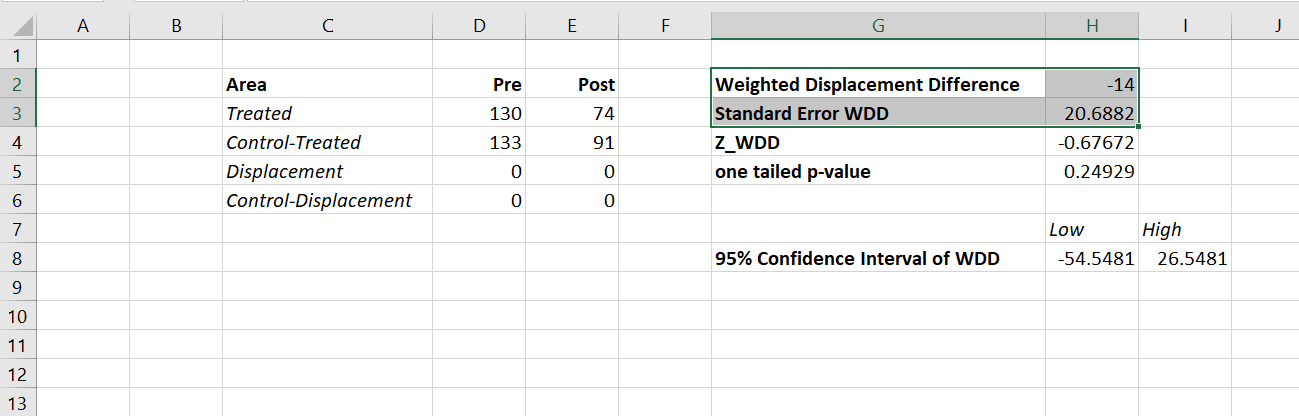Then you want to place those point estimates and standard errors in a new table, and in those same rows assign your arbitrary weight. Here I use weights taken from Ratcliffe (2015), but these weights can be anything. See examples in Wheeler & Reuter (2020) for using police cost of crime estimates, and Wolfgang et al. (2006) for using surveys on public perceptions of severity. Many of the different indices though use sentencing data to derive the weights. (You could even use negative weights and the calculations here all work, say you had some positive data on community interactions.)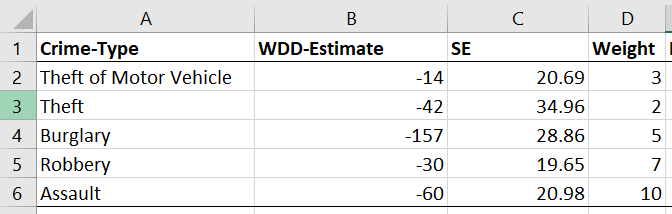Now we have all we need to calculate the harm-weighted WDD test. The big thing here to note is that the variance of `Var(x*harm_weight) = Var(x)*harm_weight^2`. So that allows me to use all the same machinery as the original WDD paper to combine all the weights in the end. So now you just need to add a few additional columns to your spreadsheet. The point estimate for the harm reduction is simply the weight multiplied by the point estimate for the crime reduction. The variance though you need to square the standard error, and square the weight, and then multiply those squared results together.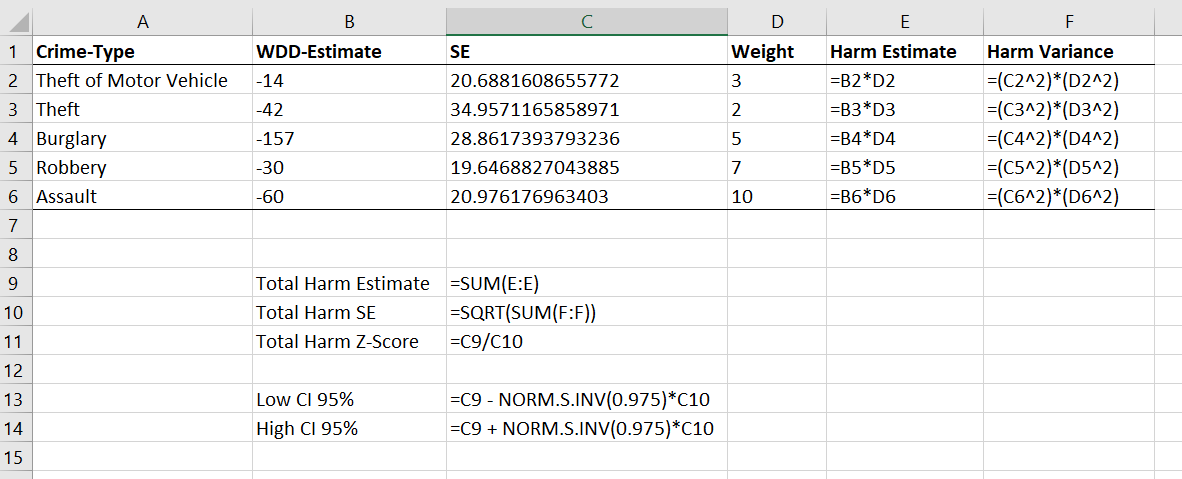Once that is done, you can pool the harm weighted stats together, see the calculations below the table. Then you can use all the same normal distribution stuff from your intro stats class to calculate z-scores, p-values, and confidence intervals. Here are what the results look like for this particular example.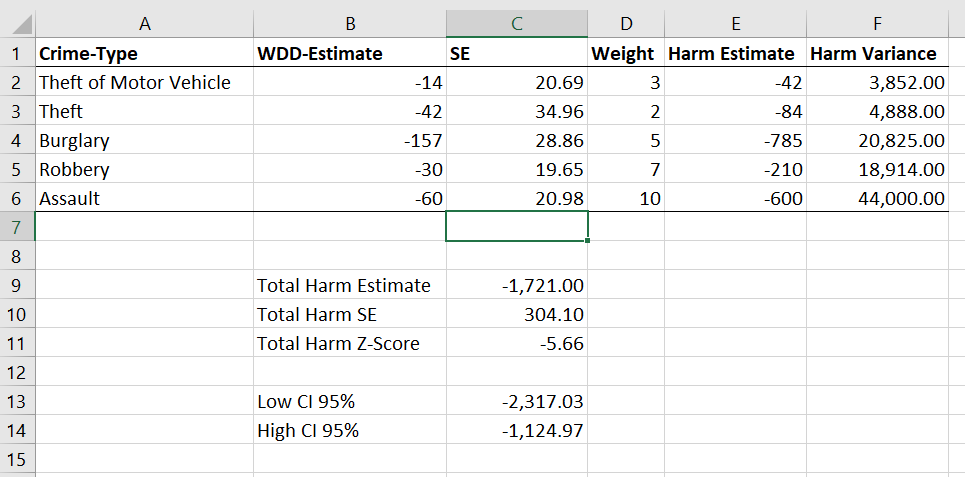I think this is actually a really good idea to pool results together. Many place based police interventions are general, in that you might expect them to reduce multiple crime types. Various harm scores are a good way to pool the results, instead of doing many individual tests. A few caveats though, I have not done simulations like I did in the WDD peer reviewed paper, I believe these normal approximations will do OK under the same circumstances though that we suggest it is reasonable to do the WDD test. You should not do the WDD test if you only have a handful of crimes in each area (under 5 in any cell in that original table is a good signal it is too few of crimes).

These crime count recommendations I think are likely to work as well for weighted crime harm. So even if you give murder a really high weight, if you have fewer than 5 murders in any of those original cells, I do not think you should incorporate it into the analysis. The large harm weight and the small numbers do not cancel each other out! (They just make the normal approximation I use likely not very good.) In that case I would say only incorporate individual crimes that you are OK with doing the WDD analysis to begin with on their own, and then pool those results together.

Sometime I need to grab the results of the hot spots meta-analysis by Braga and company and redo the analysis using this WDD estimate. I think the recent paper by Braga and Weisburd (2020) is right, that modeling the IRR directly makes more sense (I use the IRR to do cost-benefit analysis estimates, not Cohen’s D). But even that is one step removed, so say you have two incident-rate-ratios (IRRs), 0.8 and 0.5, the latter is bigger right? Well, if the 0.8 study had a baseline of 100 crimes, that means the reduction is `100 - 0.8*100 = 20`, but if the 0.5 study had a baseline of 30 crimes, that would mean a reduction of `30 - 0.5*30 = 15`, so in terms of total crimes is a smaller effect. The WDD test intentionally focuses on crime counts, so is an estimate of the actual number of crimes reduced. Then you can weight those actual crime decreases how you want to. I think worrying about the IRR could even be one step too far removed.

# A latent variable approach to RTM using hidden layers in deep learning

Sorry about the long title! Previously I have blogged about how to use Deep Learning to generate an RTM like model variable selection and positive constraints. Deep learning frameworks often do not rely on variable selection like that though, they more often leverage hidden layers. For social scientists familiar with structural equation modelling, these hidden layers are very much akin to formative latent variables. (More traditionally folks use reflective latent variables in factor analysis, so the latent variable causes the observed measures. This is the obverse, the observed measures cause/define the latent variable, and we find the loadings that best predict some outcome further down the stream.)

In a nutshell, instead of the typical RTM way of picking the best variable to use, e.g. `Alcohol Density < 100 meters` OR `Alcohol Density < 500 meters`, it allows both to contribute to a latent variable, call it `AlcoholDens`, but allows those weights to vary. Then I see how well the `AlcoholDens` latent variable predicts crime. I will show later in the results that the loadings are often spread out among different density/distance measures in this sample, suggesting the approach just pick one is perhaps misguided.

I’ve posted the data and code to follow along here. There are two py files, `00_RTMHidden.py` runs the main analysis, but `dl_rtm_funcs.py` has various functions used to build the deep learning model in pytorch. I am just going to hit some of the highlights instead of walking through bit by bit.

# Some helper functions

First, last blog post I simply relied on using Poisson loss. This time, I took some effort to figure out my own loss function for the negative binomial model. Here I am using the NB2 form, and you can see I took the likelihood function from the Stata docs (they are a really great reference for various regression model info). To incorporate this into your deep learning model, you need to add a single parameter in your model, here I call it `disp`.

``````#Log likelihood taken from Stata docs, pg 11
#https://www.stata.com/manuals13/rnbreg.pdf
def nb2_loss(actual, log_pred, disp):
m = 1/disp.exp()
mu = log_pred.exp()
p = 1/(1 + disp.exp()*mu)
nll = torch.lgamma(m + actual) - torch.lgamma(actual+1) - torch.lgamma(m)
nll += m*torch.log(p) + actual*torch.log(1-p)
return -nll.mean()``````

A second set of helper functions I will illustrate at the end of the post is evaluating the fit for Poisson/Negative Binomial models. I’ve discussed these metrics before, they are just a python rewrite of older SPSS code I made.

``````def pred_nb(mu, disp, int_y):
inv_disp = 1/disp
p1 = gamma(int_y + inv_disp) / ( factorial(int_y)*gamma(inv_disp) )
p2 = ( inv_disp / (inv_disp + mu) ) ** inv_disp
p3 = ( mu / (inv_disp + mu) ) ** int_y
pfin = p1*p2*p3
return pfin

def nb_fit(mu, obs, disp, max_y):
res = []
cum_fit = mu - mu
for i in range(max_y+1):
pred_fit = pred_nb(mu=mu, disp=disp, int_y=i)
pred_obs = (obs == i)
res.append( (str(i), pred_obs.mean(), pred_fit.mean(), pred_obs.sum(), pred_fit.sum()) )
cum_fit += pred_fit
fin_fit = 1 - cum_fit
fin_obs = (obs > max_y)
res.append( (str(max_y+1)+'+', fin_obs.mean(), fin_fit.mean(),
fin_obs.sum(), fin_fit.sum()) )
dat = pd.DataFrame(res, columns=['Int','Obs','Pred','ObsN','PredN'])
return dat``````

# Main Analysis

Now onto the main analysis. Skipping the data loading (it is near copy-paste from my prior RTM Deep Learning post), here are the main guts to building and fitting the RTM model.

``````model = dl_rtm_funcs.RTM_hidden(gen_list=[alc_set,metro_set,c311_set],
gen_names=['AlcOutlets','MetroEntr','Dens311'])
optimizer = torch.optim.Adam(model.parameters(), lr=0.001) #1e-4

for t in range(5001):
#Forward pass
y_pred = model(comb_ten)
#Loss
loss_insample = dl_rtm_funcs.nb2_loss(y_ten, y_pred, model.dispersion)
optimizer.zero_grad()
loss_insample.backward() #retain_graph=True
optimizer.step()
if t % 100 == 0:
loss_out = dl_rtm_funcs.nb2_loss(out_ten, y_pred, model.dispersion)
print(f'iter {t}: loss in = {loss_insample.item():.5f}, loss out = {loss_out.item():.5f}')``````

And in terms of iterations, on my machine this takes less than 20 seconds to do the 5000 iterations, and it has clearly peaked out by then (both in sample 2011 and out of sample 2012).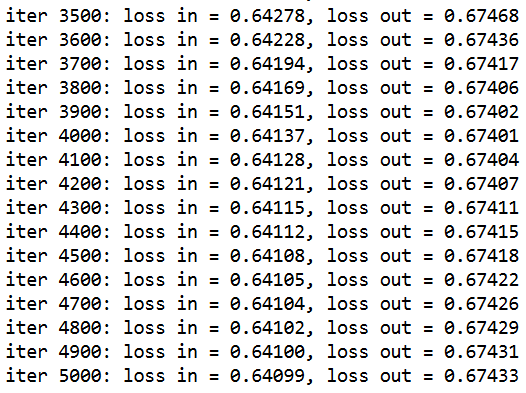I’ve loading the RTM model object with a few helper functions, so if you then run `print( model.coef_table() )`, you get out the final regression coefficients, including the dispersion term. For my negative binomial models for my dissertation, the dispersion term tended to be around ~4 for many models, so this corresponds pretty closely with my prior work.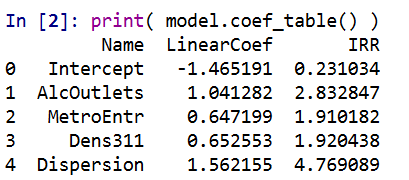These have interpretations as latent variables representing the effect of nearby alcohol outlets (both distance and density), metro entrances (just distance), and 311 calls for service (just density). Similar to original RTM, I have restricted the crime generator effects to be positive.

I also have another helper function, `model.loadings()`, that gives you a nice table. Here this shows how the original variables contribute to the latent variable. So here are the loadings for the distance to the nearest metro.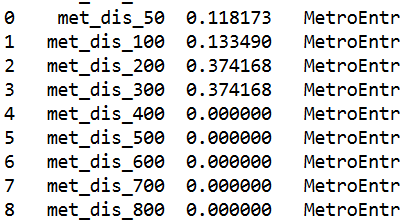You can see that the dummy variables for `met_dis_300` (meters) and smaller all contribute to the latent variable. So instead of picking one variable in the end, it allows multiple variables to contribute to the latent risk score. It may make more sense in this set up to encode variables as not cumulative, e.g. `< 50 meters, < 100 meters`, but orthogonal, e.g. `[0,50),[50,100)`, etc.), but just stuck with the prior data in the same format for now. I force the loadings to sum to 1 and be positive, so the latent variables still have a very apples-to-apples comparison in terms of effect sizes.

Here are the loadings for alcohol outlets, so we have both some distance and density effects in the end.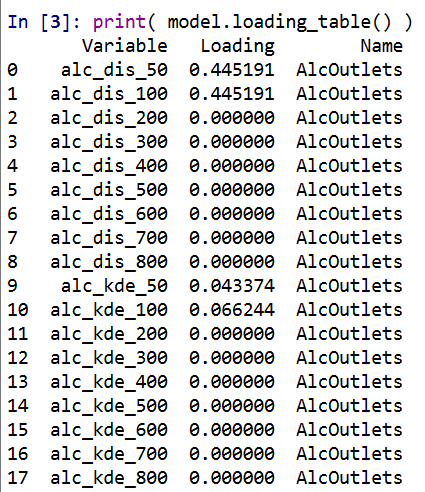And here are the loadings for 311 density variables: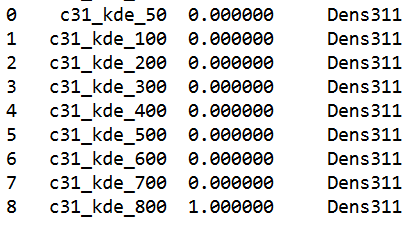So you can see for the last one, only the furthest away had an effect at all. Which is contra to the broken windows theory! But also shows that this is more general than the original RTM approach. If it only should be one variable the model will learn that, but if it should be more it will incorporate a wider array of weights.

Next is to check out how well the model does overall. For calibration for Poisson/Negative Binomial models, I just detach my pytorch tensors, and feed them into my functions to do the evaluations.

``````#Calibration for Negative Binomial predictions
pred_pd = pd.Series( y_pred.exp().detach().numpy() )
disp_val = model.dispersion.exp().item()

nb_fit = dl_rtm_funcs.nb_fit(mu=pred_pd, obs=crime_data['Viol_2011'],
disp=disp_val, max_y=10)
print( nb_fit )``````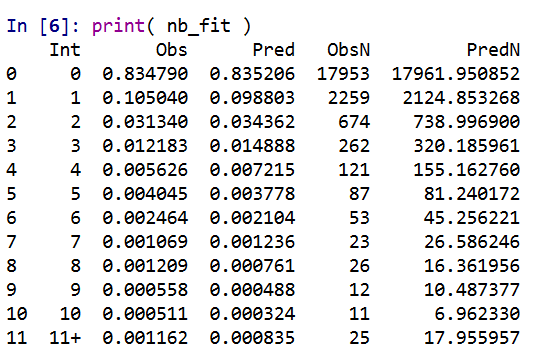So this shows that the model is pretty well calibrated in terms of overall predictions. Both samples predict 83% zeroes. I predict a few more 3/4 crime areas than observed, and my tails are somewhat thinner than they should be, but only by a tiny bit. (No doubt this would improve if I incorporated more covariates, kept it simple to debug on purpose.)

We can ignore the negative binomial dispersion term and see what our model would predict in the usual Poisson case (the mean functions are the same, it is just changing the variance). To do this, just pass in a dispersion term of 1.

``````pois_fit = dl_rtm_funcs.nb_fit(mu=pred_pd, obs=crime_data['Viol_2011'],
disp=1, max_y=10)
print( pois_fit )``````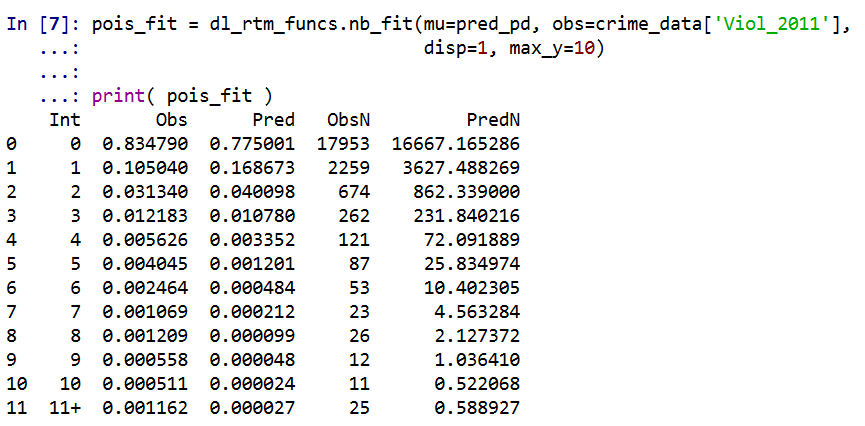You can see that the Poisson model is a much worse fit. Underpredicting zero crime areas by 6%, and areas with over 10 crimes should pretty much never happen according to the Poisson model.

We should be assessing these metrics out of sample as well, and you can see that given crime is very historically stable, the out of sample 2012 violent crime counts are similarly well calibrated.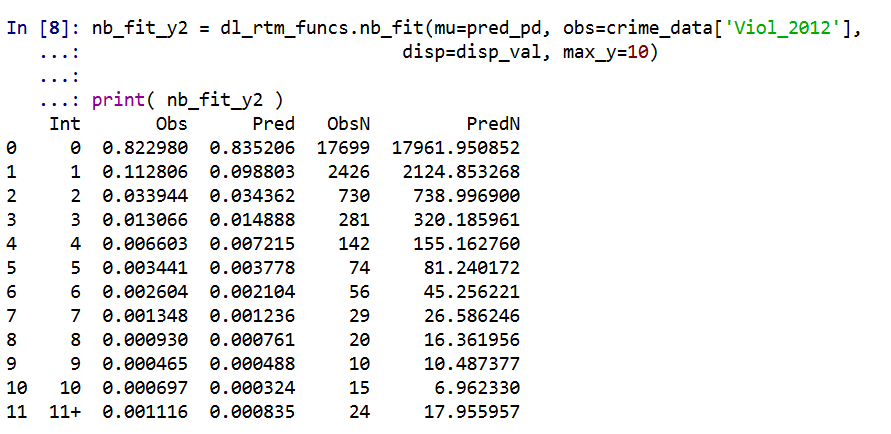Finally, I have suggested in the past to use a weighted ROC curve as a metric for crime counts. Here is a simple example of doing that in python.

``````crime_data['Weights'] = crime_data['Viol_2012'].clip(1)
crime_data['Outcome'] = crime_data['Viol_2012'].clip(0,1)

fpr, tpr, thresh = roc_curve(crime_data['Outcome'], pred_pd, sample_weight=crime_data['Weights'])
weighted_auc = auc(fpr, tpr)
print( weighted_auc ) ``````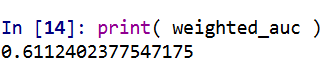So you can see the AUC is nothing to brag about here, 0.61 (it is only 0.63 in the 2011 sample). But again I am sure I could get that up by quite a bit by incorporating more covariates into the model.

• ## Follow Blog via Email

Enter your email address to follow this blog and receive notifications of new posts by email.

Join 257 other followers